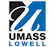Background to Ionospheric Sounding

General

The temporal and spatial variation in ionospheric structures have often frustrated the efforts of communications and radar system operators who base their frequency management decisions on monthly mean predictions of radio propagation in the high frequency (short-wave) band. The University of Massachusetts Lowell’s Center for Atmospheric Research (UMLCAR) has produced a low power miniature version of its DigisondeTM sounders, the DigisondeTM Portable Sounder (DPS), capable of making measurements of the overhead ionosphere and providing real-time on-site processing and analysis to characterize radio signal propagation to support communications or surveillance operations.

The system compensates for a low power transmitter (300 W vs. 10 kW for previous systems) by employing intrapulse phase coding, digital pulse compression and Doppler integration. The data acquisition, control, signal processing, display, storage and automatic data analysis functions have been condensed into a single multi-tasking, multiple processor computer system, while the analog circuitry has been condensed and simplified by the use of reduced transmitter power, wide bandwidth devices, and commercially available PC expansion boards. The DPS is shown in the composite Figure 1-1 (with the integrated transceiver package shown in Figure 1-1A, and one of the four crossed magnetic dipole receive antennas in Figure 1-1B).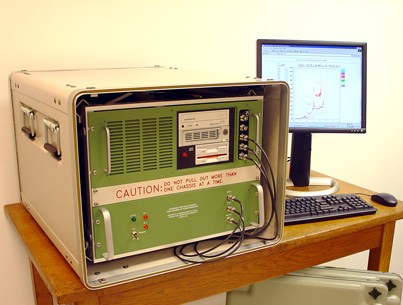Figure 1-1A DigisondeTM Portable Sounder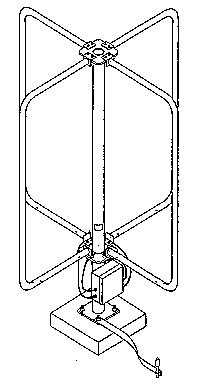Figure 1-1B Magnetic Loop Turnstile Antenna

Noteworthy new technology involved in this system includes:

• Electronically switched active crossed loop receiving antenna
• Commercially sourced 10 MIPS TMS 320C25 digital signal processor (DSP)
• 4 million sample DSP buffer memory
• 71 to 110 MHz digital synthesizer on a 4"x5" card
• Compact DC-DC converters allowing operation on one battery
• Four-channel high speed (1 million 12-bit samples/sec) digitizer board
• A 160 Mbits/sec parallel data bus between the digitizer and the DSP
• A proprietary multi-tasking operating system for remote interaction via a modem connection without suspending system operation
• Direct digital synthesized coherent oscillators
• 21 dB signal processing gain from phase coded pulse compression
• 21 dB additional signal processing gain from coherent Doppler integration
• Automatic ionospheric layer identification and parameter scaling by an embedded expert system

The availability of a small low power ionosonde that could be operated on-site wherever a high frequency (HF) radio or radar was in use, would greatly increase the value of the information produced by the instrument since it would become available to the end user immediately.

One of the chief applications for the real-time data currently provided by digital ionospheric sounders is to manage the operation of HF radio channels and networks. Since many HF radios are operated at remote locations (i.e., aircraft, boats, land vehicles of all sorts, and remote sites where telephone service is unreliable) the major obstacle to making practical use of the ionospheric sounder data and associated computed propagation information is the dissemination of this data to a data processing and analysis site. Since HF is often used where no alternative communications link exists, or is held in reserve in case primary communication is lost, it is not practical to assume that a communications link exists to make centrally tabulated real-time ionospheric data available to the user. Furthermore, local measurements are superior to measurements at sites of opportunity in the user’s general region of the globe since extreme variations in ionospheric properties are possible even over short distances, especially at high latitudes [Buchau et al., 1985; Buchau and Reinisch, 1991] or near the sunset or sunrise terminator.

However, for most applications, the size, weight, power consumption and cost of a conventional ionospheric sounder have made local measurements impractical. Therefore the availability of a small, low cost sounder is a major improvement in the usefulness of ionospheric sounder data. Shrinking the conventional 1 to 50 kW pulse sounders to a portable, battery operated 100 to 500 W system requires the application of substantial signal processing gain to compensate for the 20 dB reduction in transmitter power. Furthermore, a compact portable package requires the use of highly integrated control, data acquisition, timing, data processing, display and storage hardware.

The objective of the DPS development project was to develop a small vertical incidence (i.e., monostatic) ionospheric sounder which could automatically collect and analyze ionospheric measurements at remote operating sites for the purpose of selecting optimum operating frequencies for obliquely propagated communication or radar propagation paths. Intermediate objectives assumed to be necessary to produce such a capability were the development of optimally efficient waveforms and of functionally dense signal generation, processing and ancillary circuitry. Since the need for an embedded general purpose computer was a given imperative, real-time control software was developed to incorporate as many functions as was feasible into this computer rather than having to provide additional circuitry and components to perform these functions. The DPS duplicates all of the functions of its predecessor the DigisondeTM 256 [Bibl et al., 1981] and [Reinisch, 1987] in a much smaller, low power package. These include the simultaneous measurement of seven observable parameters of reflected (or in oblique incidence, refracted) signals received from the ionosphere:

1) Frequency
2) Range (or height for vertical incidence measurements)
3) Amplitude
4) Phase
6) Angle of Arrival
7) Wave Polarization

Because the physical parameters of the ionospheric plasma affect the way radio waves reflect from or pass through the ionosphere, it is possible by measuring all of these observable parameters at a number of discrete heights and discrete frequencies to map out and characterize the structure of the plasma in the ionosphere. Both the height and frequency dimensions of this measurement require hundreds of individual measurements to approximate the underlying continuous functions. The resulting measurement is called an ionogram and comprises a seven dimensional measurement of signal amplitude vs. frequency and vs. height as shown in Figure 1-2 (due to the limitations of current software only five may be displayed at a time). Figure 1-2 is a five-dimensional display, with sounding frequency as the abscissa, virtual reflection height (simple conversion of time delay to range assuming propagation at 3x108 m/sec) as the ordinate, signal amplitude as the spot (or pixel) intensity, Doppler shift as the color shade and wave polarization as the color group (the blue-green-grey scale or "cool" colors showing extraordinary polarization, the red-yellow-white scale or "hot" colors showing ordinary polarization).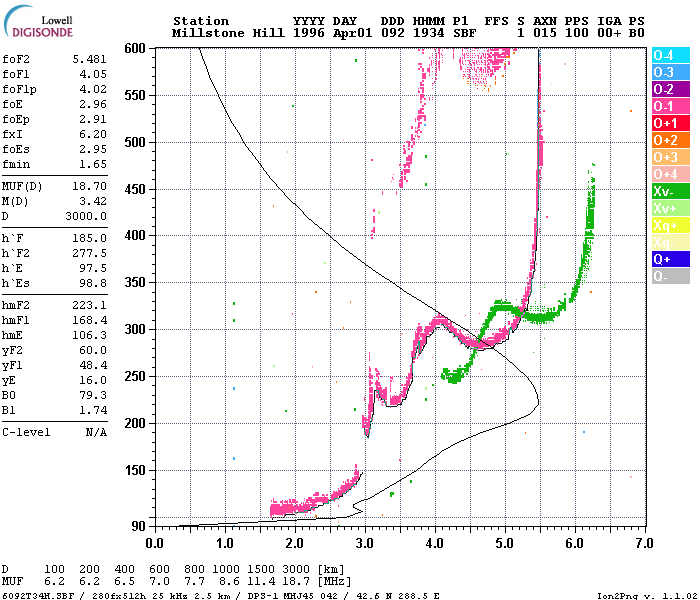Figure 1-2 Five-Dimensional Ionogram

Another objective of the DPS development was to store the data created by the system in an easily accessible format (e.g., DOS formatted personal computer files), while maintaining compatibility with the existing base of DigisondeTM sounder analysis software in use at the UMLCAR and at over 40 research institutes around the world. This objective often competed with the additional objective of providing an easily accessible and simply understood standard data format to facilitate the development of novel post-processing analysis and display programs.

An ionospheric sounder uses basic radar techniques to detect the electron density (equal to the ion density since the bulk plasma is neutral) of ionospheric plasma as a function of height. The ionospheric plasma is created by energy from the sun transferred by particles in the solar wind as well as direct radiation (especially ultra-violet and x-rays). Each component of the solar emissions tends to be deposited at a particular altitude or range of altitudes and therefore creates a horizontally stratified medium where each layer has a peak density and to some degree, a definable width, or profile. The shape of the ionized layer is often referred to as a Chapman function [Davies, 1989] which is a roughly parabolic shape somewhat elongated on the top side. The peaks of these layers usually form between 70 and 300 km altitude and are identified by the letters D, E, F1 and F2, in order of their altitude.

By scanning the transmitted frequency from 1 MHz to as high as 40 MHz and measuring the time delay of any echoes (i.e., apparent or virtual height of the reflecting medium) a vertically transmitting sounder can provide a profile of electron density vs. height. This is possible because the relative refractive index of the ionospheric plasma is dependent on the density of the free electrons (Ne), as shown in Equation 1-1 (neglecting the geomagnetic field):

m2(h)= 1 – k (Ne/f2) (1–1)

where k = 80.5, Ne is electrons/m3, and f is in Hz [Davies, 1989; Chen, 1987].

The behavior of the plasma changes significantly in the presence of the Earth’s magnetic field. An exhaustive derivation of m [Davies, 1989] results in the Appleton Equation for the refractive index, which is one of the fundamental equations used in the field of ionospheric propagation. This equation clearly shows that there are two values for refractive index, resulting in the splitting of a linearly polarized wave incident upon the ionosphere, into two components, known as the ordinary and extraordinary waves. These propagate with a different wave velocity and therefore appear as two distinct echoes. They also exhibit two distinct polarizations, approximately right hand circular and left hand circular, which aid in distinguishing the two waves.

When the transmitted frequency is sufficient to drive the plasma at its resonant frequency there is a total internal reflection. The plasma resonance frequency (fp) is defined by several constants, e – the charge of an electron, m – the mass of an electron, eo – the permittivity of free space, but only one variable, Ne – electron density in electrons/m3 [Chen, 1987]:

fp2 = (Ne e2/4peom) = kNe                         (1-2)

A typical number for the F-region (200 to 400 km altitude) is 1012 electrons/m3, so the plasma resonance frequency would be 9 MHz. The value of m in Equation 1–1 approaches 0 as the operating frequency, f, approaches the plasma frequency. The group velocity of a propagating wave is proportional to m, so m = 0 implies that the wave slows down to zero which is obviously required at some point in the process of reflection since the propagation velocity reverses.

The total internal reflection from the ionosphere is similar to reflection of radio frequency (RF) energy from a metal surface in that the re-radiation of the incident energy is caused by the free electrons in the medium. In both cases the wave penetrates to some depth. In a plasma the skin depth (the depth into the medium at which the electric field is 36.8% of its incident amplitude) is defined by:

l0/2p
d =  ----------			                (1-3)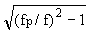where l0 is the free space wavelength.

The major difference between ionospheric reflection and reflection from a metallic surface is that the latter has a uniform electron density while the ionospheric density increases roughly parabolically with altitude, with densities starting at essentially zero at stratospheric altitudes and rising to a peak at about 200 to 400 km. In the case of a metal there is no region where the wave propagates below the resonance frequency, while in the ionosphere the refractive index and therefore the wave velocity change with altitude until the plasma resonance frequency is reached. Of course if the RF frequency is above the maximum plasma resonance frequency the wave is never reflected and can penetrate the ionosphere and propagate into outer space. Otherwise what happens on a microscopic scale at the surface of a metal and on a macroscopic scale at the plasma resonance in the ionosphere is very similar in that energy is re-radiated by electrons which are responding to the incident electric field.

During the 1960’s and 1970’s several variations in sounding techniques started moving significantly beyond the basic pulse techniques developed in the 1930’s. First was the coherent integration of several pulses transmitted at the same frequency. Two signals are coherent if, having a phase and amplitude, they are able to be added together (e.g., one radar pulse echo received from a target added to the next pulse echo received from the same target, thousandths of a second later) in such a way that the sum may be zero (if the two signals are exactly out of phase with each other) or double the amplitude (if they are exactly in phase). Coherent integration of N signals can provide a factor of N improvement in power. This technique was first used in the DigisondeTM 128 [Bibl and Reinisch, 1975].

In ionospheric sounding, the motion of the ionosphere often makes it impossible to integrate by simple coherent summation for longer that a fraction of a second, although it is not rare to receive coherent echoes for tens of seconds. However, with the application of spectral integration (which is a byproduct of the Fourier transform used to create a Doppler spectrum) it is possible to coherently integrate pulse echoes for tens of seconds under nearly all ionospheric conditions [Bibl and Reinisch, 1978]. The integration may progress for as long a time as the rate of change of phase remains constant (i.e., there is a constant Doppler shift, Df). The DigisondeTM 128PS, and all subsequent versions perform this spectral integration.

Additional detail on this topic is contained in Chapter 2 in this section.

A third general technique to improve on the simple pulse sounder is to stretch out the pulse by a factor of N, thus increasing the duty cycle so the pulse contains more energy without requiring a higher power transmitter (power x time = energy). However, to maintain the higher range resolution of the simple short pulse the pulse can be bi-phase, or phase reversal modulated with a phase code to enable the receiver to create a synthetic pulse with the original (i.e., that of the short pulse) range resolution. A network of sounders using a 13-bit Barker Code were operated by the U.S. Navy in the 1960’s.

The critical factor in the use of pulse compression waveforms for any radar type measurement is the correlation properties of the internal phase code. Phase codes proposed and experimented with included the Barker Code [Barker, 1953], Huffman Sequences [Huffman 1962], Convoluted Codes [Coll, 1961], Maximal Length Sequence Shift Register Codes (M-codes) [Sarwate and Pursley, 1980], or Golay’s Complementary Sequences [Golay, 1961], which have been implemented in the VHF mesospheric sounding radar at Ohio State University [Schmidt et al., 1979] and in the DPS. The internal phase code alternative has just recently become economically feasible with the availability of very fast microprocessor and signal processor IC’s. Barker Coded pulses have been implemented in several ionospheric sounders to date, but until the DPS was developed there have been no other successful implementations of Complementary Series phase codes in ionospheric sounders.

The European Incoherent Scatter radar in Tromso, Norway (VanEiken, 1991 and 1993) and an over-the-horizon (OTH) HF radar used the Complementary Series codes. However most major radar systems including all currently active OTH radars opted for the FM/CW chirp technique, due to its resistance to Doppler induced leakage and its compatibility with analog pulse compression processing techniques. Basically, the chirp waveform avoids the need for extremely fast digital processing capabilities, since only the final stage is performed digitally, while the pulse compression is best performed entirely digitally. Even at the modest bandwidths used for ionospheric sounding, this digital capability was until recently, much more expensive and cumbersome than the special synthesizers required for chirpsounding.

Another new development in the 1970’s was the coherent multiple receiver array [Bibl and Reinisch, 1978] which allows angle of arrival (incidence angle) to be deduced from phase differences between antennas by standard interferometer techniques. Given a known operating frequency, and known antenna spacing, by measuring the phase or phase difference on a number of antennas, the angle of arrival of a plane wave can be deduced. This interferometry solution is invalid, however, if there are multiple sources contributing to the received signal (i.e., the received wave therefore does not have a planar phase front). This problem can be overcome in over 90% of the cases as was first shown with the DigisondeTM 256 [Reinisch et al., 1987] by first isolating or discriminating the multiple sources in range, then in the Doppler domain (i.e., isolating a plane wavefront) before applying the interferometry relationships.

Except for the FM/CW chirpsounder which operates well on transmitter power levels of 10 to 100 W (peak power) the above techniques and cited references typically employ a 2 to 30 kW peak power pulse transmitter. This power is needed to get sufficient signal strength to overcome an atmospheric noise environment which is typically 20 to 50 dB (CCIR Noise Tables) above thermal noise (defined as kTB, the theoretical minimum noise due to thermal motion, where k = Boltzman’s constant, T = temperature in ° K, and B = system bandwidth in Hz). More importantly, however, since ionogram measurements require scanning of the entire propagating band of frequencies in the 0.5 to 20 MHz RF band (up to 45 MHz for oblique measurements), the sounder receiver will encounter broadcast stations, ground-to-air communications channels, HF radars, ship-to-shore radio channels and several very active radio amateur bands which can add as much as 60 dB more background interference. Therefore, the sounder signal must be strong enough to be detectable in the presence of these large interfering signals.

To make matters worse, a pulse sounder signal must have a broad bandwidth to provide the capability to accurately measure the reflection height, therefore the receiver must have a wide bandwidth, which means more unwanted noise is received along with the signal. The noise is distributed quite evenly over bandwidth (i.e., white), while interfering signals occur almost randomly (except for predictably larger probabilities in the broadcast bands and amateur radio bands) over the bandwidth. Thus a wider-bandwidth receiver receives proportionally more uniformly distributed noise and the probability of receiving a strong interfering signal also goes up proportionally with increased bandwidth.

The DPS transmits only 300 W of pulsed RF power but compensates for this low power by digital pulse compression and coherent spectral (Doppler) integration. The two techniques together provide about 30 dB of signal processing gain (up to 42 dB for the bi-static oblique waveforms) thus for vertical incidence measurements the system performs equivalently with a simple pulse sounder of 1000 times greater power (i.e., 300 kW).

Additional detail on this topic is contained in Chapter 2 in this section.

Current applications of ionospheric sounders fall into two categories:

a. Support of operational systems, including shortwave radio communications and OTH radar systems. This support can be in the form of predictions of propagating frequencies at given times and locations in the future (e.g., over the ensuing month) or the provision of real-time updates (updated as frequently as every 15 minutes) to detect current conditions such that system operating parameters can be optimized.

b. Scientific research to enable better prediction of ionospheric conditions and to understand the plasma physics of the solar-terrestrial interaction of the Earth’s atmosphere and magnetic field with the solar wind.

There has been considerable effort in producing global models of ionospheric densities, temperature, chemical constitution, etc, such that a few sounder measurements could calibrate the models and improve the reliability of global predictions. It has been shown that if measurements are made within a few hundred kilometers of each other, the correlation of the measured parameters is very high [Rush, 1978]. Therefore a network of sounders spaced by less than 500 km can provide reliable estimates of the ionosphere over a 250 km radius around them.

The areas of research pursued by users of the more sophisticated features of the DigisondeTM sounders include polar cap plasma drift, auroral phenomena, equatorial spread-F and plasma irregularity phenomena, and sporadic E-layer composition [Buchau et al., 1985; Reinisch 1987; and Buchau and Reinisch 1991]. There may be some driving technological needs (e.g., commercial or military uses) in some of these efforts, but many are simply basic research efforts aimed at better understanding the manifestations of plasma physics provided by nature.

The detailed design and synthesis of a RF measurement system (or any electronic system) must be based on several criteria:

a. The performance requirements necessary to provide the needed functions, in this case scientific measurements of electron densities and motions in the ionosphere.

b. The availability of technology to implement such a capability.

c. The cost of purchasing or developing such technology.

d. The risk involved in depending on certain technologies, especially if some of the technology needs to be developed.

e. The capabilities of the intended user of the system, and its expected willingness to learn to use and maintain it; i.e., how complicated can the operation be before the user will give up and not try to learn it.

The question of what technology can be brought to bear on the realization of a new ionospheric sounder was answered in a survey of existing technology in 1989, when the portable sounder development started in earnest. This survey showed the following available components, which showed promise in creating a smaller, less costly, more powerful instrument. Many of these components were not available when the last generation of DigisondesTM (circa 1980) was being developed:

Solid-state 300 W MOSFET RF power transistors

High-speed high precision (12, 14 and 16 bit) analog to digital (A–D) converters

High-speed high precision (12 and 16 bit) digital to analog (D–A) converters

Single chip Direct Digital Synthesizers (DDS)

Wideband (up to 200 MHz) solid state op amps for linear feedback amplifiers

Wideband (4 octaves, 2–32 MHz) 90° phase shifters

Proven DigisondeTM 256 measurement techniques

Very fast programmable DSP (RISC) IC’s

Fast, single board, microcomputer systems and supporting programming languages

Many of these components are inexpensive and well developed because they feed a mass market industry. The MOSFET transistors are used in Nuclear Magnetic Resonance medical imaging systems to provide the RF power to excite the resonances. The high speed D–A converters are used in high resolution graphic video display systems such as those used for high performance workstations. The DDS chips are used in cellular telephone technology, in which the chip manufacturer, Qualcomm, is an industry leader. The DSP chips are widely used in speech processing, voice recognition, image processing (including medical instrumentation). And of course, fast microcomputer boards are used by many small systems integrators which end up in a huge array of end user applications ranging from cash registers to scientific computing to industrial process controllers.

The performance parameters were well known at the beginning of the DPS development, since several models of ionospheric pulse sounders had preceded it. The frequency range of 1 to 20 MHz for vertical sounding was an accepted standard, and 2 to 30 MHz was accepted as a reasonable range for oblique incidence measurements. It was well known that radio waves of greater than 30 MHz often do propagate via skywave paths, however, most systems relying on skywave propagation don’t support these frequencies, so interest in this frequency band would only be limited to scientific investigations. A required power level in the 5 to 10 kW range for pulse transmitters had provided good results in the past. The measurement objectives were to simultaneously measure all seven observable parameters outlined at Paragraph 107 above in order to characterize the following physical features:

The height profile of electron density vs. altitude

Position and spatial extent of irregularity structures, gradients and waves

Motion vectors of structures and waves

As mentioned in the section above dealing with Current Applications of Ionospheric Sounding (Paragraph 127 et seq. above), the accurate measurement of all of the parameters, except frequency (it being precisely set by the system and need not be measured) depends heavily on the signal to noise ratio of the received signal. Therefore vertical incidence ionospheric sounders capable of acquiring high quality scientific data have historically utilized powerful pulse transmitters in the 2 to 30 kW range. The necessity for an extremely good signal to noise ratio is demanded by the sensitivity of the phase measurements to the random noise component added to the signal level. For instance, to measure phase to 1 degree accuracy requires a signal to noise ratio better than 40 dB (assuming a Gaussian noise distribution which is actually a best case), and measurement of amplitude to 10% accuracy requires over 20 dB signal to noise ratio. Of course, is it desirable that these measurements be immune to degradation from noise and interference and maintain their high quality over a large frequency band. This requires that at the lower end of the HF band the system’s design has to overcome absorption, noise and interference, and poor antenna performance and still provide at least a 20 to 40 dB signal to noise ratio.

General

The VIS/DPS borrows several of the well proven measurement techniques used by the DigisondeTM 256 sounder described in [Bibl, et al, 1981; Reinisch et al., 1989] and [Reinisch, 1987], which has been produced for the past 12 years by the UMLCAR. The addition of digital pulse compression in the DPS makes the use of low power feasible, the implementation in software of processes that were previously implemented in hardware results in a much smaller physical package, and the high level language control software and standard PC-DOS (i.e., IBM/PC) data file formats provide a new level of flexibility in system operation and data processing.

A technical description of the DPS (sounder unit and receive antennas sub-systems) are contained in Section 2 of this manual.

The DPS is able to be miniaturized by lengthening the transmitted pulse beyond the pulse width required to achieve the desired range resolution where the radar range resolution is defined as,

DR=c/2b       where b is the system bandwidth, or                                    (1-4)

DR=cT/2        for a simple rectangular pulse
waveform, with T being the width
of the rectangular pulse

The longer pulse allows a small low voltage solid state amplifier to transmit an amount of energy equal to that transmitted by a high power pulse transmitter (energy = power x time, and power = V2/R) without having to provide components to handle the high voltages required for tens of kilowatt power levels. The time resolution of the short pulse is provided by intrapulse phase modulation using programmable phase codes (user selectable and firmware expandable), the Complementary Codes, and M-codes are standard. The use of a Complementary Code pulse compression technique is described in this chapter, which shows that at 300 W of transmitter power the expected measurement quality is the same as that of a conventional sounder of about 500 kW peak pulse power.

The transmitted spread spectrum signal s(t) is a biphase (180° phase reversal) modulated pulse. As illustrated in Figure 1–3, bi-phase modulation is a linear multiplication of the binary spreading code p(t) (a.k.a. a chipping sequence, where each code bit is a "chip") with a carrier signal sin(2pf0t) or in complex form, exp[j2pf0t], to create a transmitted signal,

s(t)=p(t)exp[j2pf0t]                           (1-5)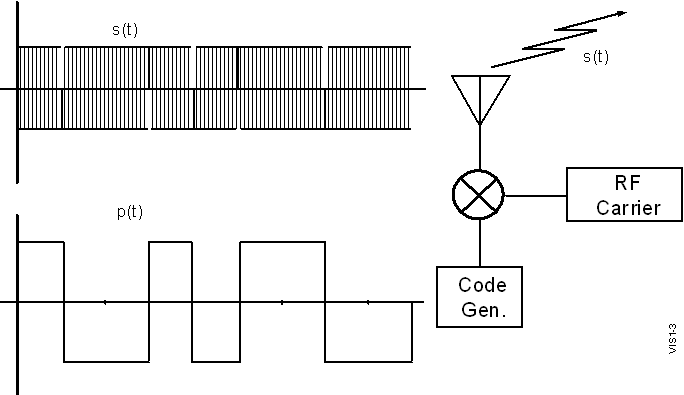Figure 1-3 Generation of a Bi-phase Modulated Spread Spectrum Waveform

NOTE

Notation throughout this chapter will use s(t) as the transmitted signal, r(t) the received signal and p(t) as the chip sequence. Functions r1(t) and r2(t) will be developed to describe the signal after various stages of processing in the receiver.

The term chip is used rather than bit because for spread spectrum communications many chips are required to transmit one bit of message information, so a distinct term had to be developed. Figure 1-4 on the following page depicts the modulation of a sinusoidal RF carrier signal by a binary code (notice that the code is a zero mean signal, i.e., centred around 0 volts amplitude). Since the mixer in Figure 1-3 can be thought of as a mathematical multiplier, the code creates a 180o (p radians) phase shift in the sinusoidal carrier whenever p(t) is negative, since –sin(wt) = sin(wt+p).

The binary spreading code is identical to a stream of data bits except that it is designed such that it forms a pattern with uniquely desirable autocorrelation function characteristics as described later in this chapter. The 16-bit Complementary Code pair used in the DPS is 1-1-0-1-1-1-1-0-1-0-0-0-1-0-1-1 modulated onto the odd-numbered pulses and 1-1-0-1-1-1-1-0-0-1-1-1-0-1-0-0 modulated onto the even-numbered pulses. This pattern of phase modulation chips is such that the frequency spectrum of such a signal (as shown in Figure 1-4) is uniformly spread over the signal bandwidth, thus the term "spread spectrum". In fact, it is interesting to note that the frequency spectrum content of the spread spectrum signal used by the DPS is identical to that of the higher peak power, simple short pulse used by the DigisondeTM 256, even though the physical pulse is 8 times longer. Since they have the same bandwidth, Equation 1–4 would suggest that they have the same range resolution. It will be shown later in this chapter, that the ability of the DigisondeTM 256 and the DPS to determine range (i.e., time delay), phase, Doppler shift and angle of arrival is also identical between the two systems, even though the transmitted waveforms appear to be vastly different.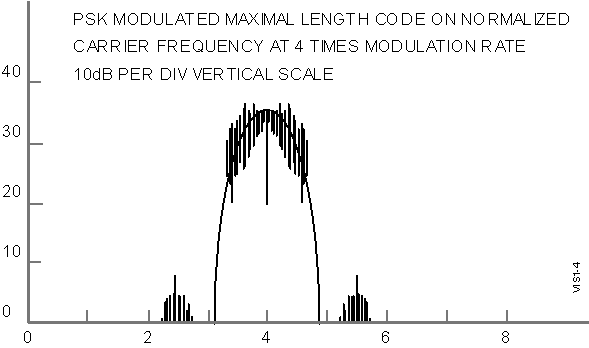Figure 1–4 Spectral Content of a Spread-Spectrum Waveform

Since the transmitted signal would obscure the detection of the much weaker echo in a monostatic system the transmitted pulse must be turned off before the first E-region echoes arrive at the receiver which, as shown in Figure 1-5, is about TE = 600 m sec after the beginning of the pulse. Also, since the receiver is saturated when the transmitter pulse comes on again, the pulse repetition frequency is limited by the longest time delay (listening interval) of interest, which is at least 5 msec, corresponding to reflections from 750 km altitude. To meet these constraints, a 533 m sec pulse made up of eight 66.67 m sec phase code chips (15 000 chips/sec) is selected which allows detection of ionospheric echoes starting at 80 km altitude. To avoid excessive range ambiguity, a highest pulse repetition frequency of 200 pps is chosen, which allows reception of the entire pulse from a virtual height of 670 km (the pulse itself is 80 km long) altitude before the next pulse is transmitted. This timing captures all but the highest multihop F-region echoes which are of little interest. Under conditions where higher unambiguous ranges, and therefore longer receiver listening intervals, are desired 100 pps or 50 pps can be selected under software control.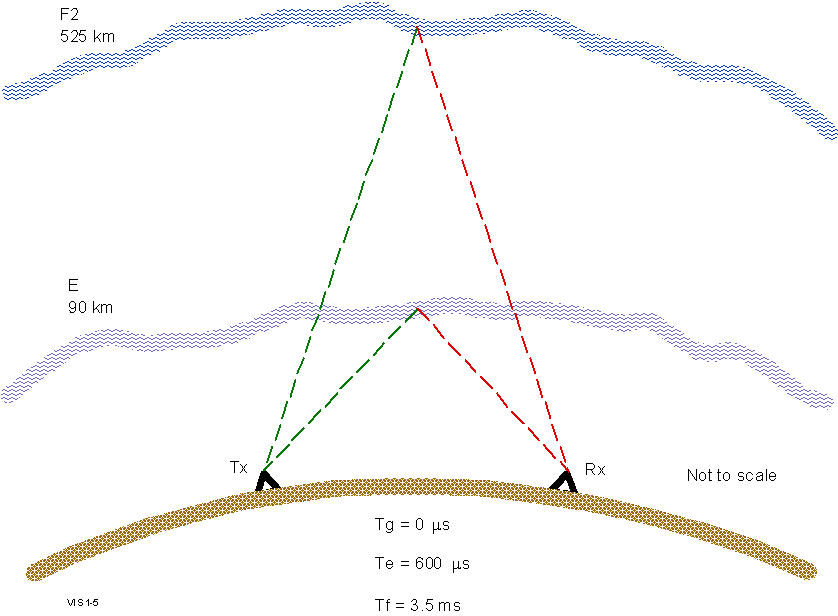Figure 1-5 Natural Timing Limitations for Monostatic Vertical Incidence Sounding

The key to the pulse compression technique lies in the selection of a spreading function, p(t), which possesses an autocorrelation function appropriate for the application. The ideal autocorrelation function for any remote sensing application is a Dirac delta function (or instantaneous impulse, d (t) since this would provide perfect range accuracy and infinite resolution. However, since the Dirac delta function has infinite instantaneous power and infinite bandwidth, the engineering tradeoffs in the design of any remote sensing system mainly involve how far one can afford to deviate from this ideal (or how much one can afford to spend in more closely approximating this ideal) and still achieve the accuracy and resolution required. More to the point, for a discussion of a discrete time digital system such as the DPS, the ideal signal is a complex unit impulse function, with the phase of the impulse conveying the RF phase of the received signal. The many different pulse compression codes all represent some compromise in achieving this ideal, although each code has its own advantages, limitations, and trade-offs. The autocorrelation function as applied to code compression in the VIS/DPS is defined as:

k
R(k)=S p(n) p(n+k)                               (1-6)
n

Therefore the ideal as described above is R(k) = d(k). (Several examples of autocorrelation functions of the codes described in this Section can be seen in Figures 1-9 through 1-13.)

For ionospheric applications, the received spread-spectrum coded signal, r(t), may be a superposition of several multipath echoes (i.e., echoes which have traveled over various propagation paths between the transmitter and receiver) reflected at various ranges from various irregular features in the ionosphere. The algorithm used to perform the code compression operates on this received multipath signal, r(t), which is an attenuated and time delayed (possibly multiple time delays) replica of the transmitted signal s(t) (from Equation 1–5), which can be represented as:

P
r(t)=S ai s(t-ti)    or                                    (1-7)
i=1
P
r(t)=S ai  p(t-ti)exp[j2pf0t - fi]
i=1

where S shows that the P multipath signals sum linearly at the receive antenna, ai is the amplitude of the ith multipath component of the signal, and ti is the propagation delay associated with multipath i. The carrier phase fi of each multipath could be expressed in terms of the carrier frequency and the time delay t i ; however, since the multiple carriers (from the various multipath components) cannot be resolved, while the delays in the complex code modulation envelope can be, a separate term, f i, is used. Next, when the carrier is stripped off of the signal, this RF phase term will be represented by a complex amplitude coefficient ai rather than ai.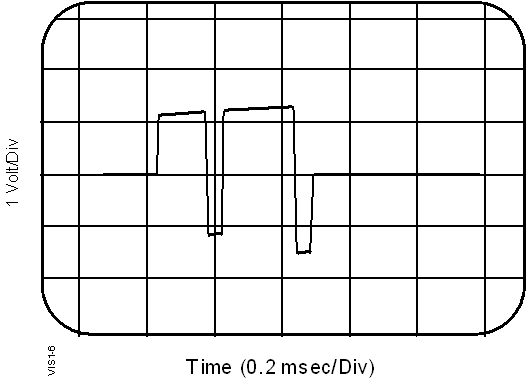Figure 1-6 Conversion to Baseband by Undersampling

By down-converting to a baseband signal (a digital technique is shown in Figure 1-6), the carrier signal can be stripped away, leaving only the superposed code envelopes delayed by P multiple propagation paths. Figure 1-6 presents one way to strip the carrier off a phase modulated signal. This is the screen display on a digital storage oscilloscope looking at the RF output from the DPS system operating at 3.5 MHz. Notice that the horizontal scan spans 2 msec, which if the oscilloscope was capable of presenting more than 14 000 resolvable points, would display 7 000 cycles of RF. The sample clock in the digital storage scope is not synchronized to the DPS, however, the digital sampling remains coherent with the RF for periods of several milliseconds. The analog signal is digitized at a rate such that each sample is made an integer number of cycles apart (i.e., at the same phase point) and therefore looks like a DC level until the phase modulation creates a sudden shift in the sampled phase point. Therefore the 180º phase reversals made on the RF carrier show up as DC level shifts, replicating the original modulating code exactly. The more hardware intensive method of quadrature demodulation with hardware components (mixers, power splitters and phase shifters) can be found in any communications systems textbook, such as [Peebles, 1979]. After removing the carrier, the modified r(t), now represented by r1(t) becomes:

P
r1(t)=S ai p(t-ti)                                       (1-8)
i=1

where the carrier phase of each of the multipath components is now represented by a complex amplitude a i which carries along the RF phase term, originally defined by f i in Equation 1–7, for each multipath. Since the pulse compression is a linear process and contributes no phase shift, the real and imaginary (i.e., in-phase and quadrature) components of this signal can be pulse compressed independently by cross-correlating them with the known spreading code p(t). The complex components can be processed separately because the pulse compression (Equation 1–9B) is linear and the code function, p(n), is all real. Therefore the phase of the cross-correlation function will be the same as the phase of r1(t).

The classical derivation of matched filter theory [e.g., Thomas, 1964] creates a matched filter by first reversing the time axis of the function p(t) to create a matched filter impulse response h(t) = p(–t). Implementing the pulse compression as a linear system block (i.e., a "black box" with impulse response h(t)) will again reverse the time axis of the impulse response function by convolving h(t) with the input signal. If neither reversal is performed (they effectively cancel each other) the process may be considered to be a cross-correlation of the received signal, r(t) with the known code function, p(t). Either way, the received signal, r2(n) after matched filter processing becomes:

r2(n)=r1(n)*h(n)=r1(n)*p(-n)                    (1-9A)

or by substituting Equation 1–8 and writing out the discrete convolution, we obtain the cross-correlation approach,

P      M                         P
r2(n)=S ai  S p(k-ti)p(k-n)=S Mai d(n-ti)              (1-9B)
i=1    k=1                i=1

where n is the time domain index (as in the sample number, n, which occurs at time t = nT where T is the sampling interval), P is the number of multipaths, k is the auxiliary index used to perform the convolution, and M is the number of phase code chips. The last expression in Equation 1–9B, the d(n), is only true if the autocorrelation function of the selected code, p(t), is an ideal unit impulse or "thumbtack" function (i.e., it has a value of M at correlation lag zero, while it has a value of zero for all other correlation lags). So, if the selected code has this property, then the function r2(n), in Equation 1–9 is the impulse response of the propagation path, which has a value ai, (the complex amplitude of multipath signal i) at each time n = t i (the propagation delay attributable to multipath I).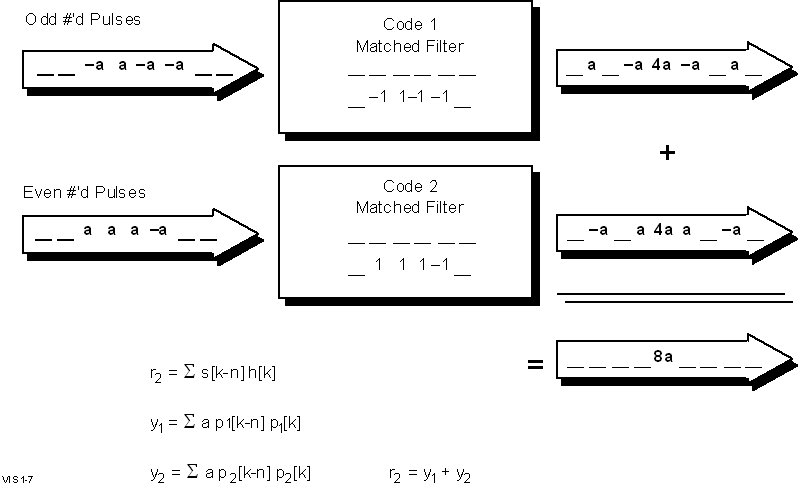Figure 1-7 Illustration of Complementary Code Pulse Compression

Figure 1-7 illustrates the unique implementation of Equation 1–9 employed for compression of Complementary Sequence waveforms. A 4-bit code is used in this figure for ease of illustration but arbitrarily long sequences can be synthesized (the DPS’s Complementary Code is 8-chips long). It is necessary to transmit two encoded pulses sequentially, since the Complementary Codes exist in pairs, and only the pairs together have the desired autocorrelation properties. Equation 1–8 (the received signal without its sinusoidal carrier) is represented by the input signal shown in the upper left of Figure 1-7. The time delay shifts (indexed by n in Equation 1–9 are illustrated by shifting the input signal by one sample period at a time into the matched filter. The convolution shifts (indexed by k in Equation 1–9 sequence through a multiply-and-accumulate operation with the four ± 1 tap coefficients. The accumulated value becomes the output function r2(n) for the current value of n. The two resulting expressions for Equation 1–9 (an r2(n) expression for each of the two Complementary Codes) are shown on the right with the amplitude M=4 clearly expressed. The non-ideal approximation of a delta function, d(n–ti), is apparent from the spurious a and –a amplitudes. However, by summing the two r2(n) expressions resulting from the two Complementary Codes, the spurious terms are cancelled, leaving a perfect delta function of amplitude 2M.

The amplitude coefficient M in Equation 1–9 is tremendously significant! It is what makes spread-spectrum techniques practical and useful. The M means that a signal received at a level of 1 mv would result in a compressed pulse of amplitude M mv, a gain of 20 log10(M) dB. Unfortunately, the benefits of all of that gain are not actually realized because the RMS amplitude of the random noise (which is incoherently summed by Equation 1–9B) which is received with the signal goes up by a factor of \/M. However, this still represents a power gain (since power = amplitude2) equal to M, or 10log10(M) dB. The \/M coefficient for the incoherent summation of multiple independent noise samples is developed more thoroughly in the following section on Coherent Spectral Integration, but the factor of M-increase for the coherent summation of the signal is clearly illustrated in Figure 1-7.

The next concern is that the pulse compression process is still valid when multiple signals are superimposed on each other as occurs when multipath echoes are received. It seems likely that multiple overlapping signals would be resolved since Equation 1–9 and the free space propagation phenomenon are linear processes, so the output of the process for multiple inputs should be the same as the sum of the outputs for each input signal treated independently. This linearity property is illustrated in Figure 1-8. Two 4-chip input signals, one three times the amplitude of the other, are overlapped by two chips at the upper left of the illustration. After pulse compression, as seen in the lower right, the two resolved components, still display a 3:1 amplitude ratio and are separated by two chip periods.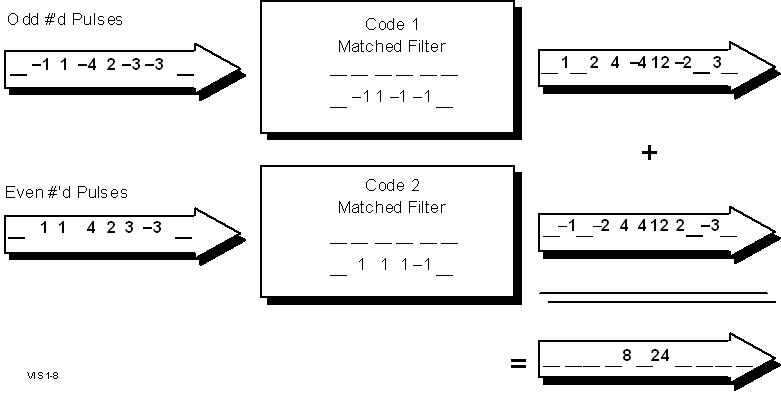Figure 1-8 Resolution of Overlapping Complementary Coded Pulses

The phase of the received signal is detected by quadrature sampling; but, how is the complex quantity, a i, or ai exp[fi], related to the RF phase (fi) of each individual multipath component? It can be shown that this phase represents the phase of the original RF signal components exactly. As shown in Equations 1–10 and 1–11, the down-converting (frequency translation) of r(t) by an oscillator, exp[j2pf0t] results in:

P                                                        P
r1(t)=Saip(t-ti)exp[j2pf0t-jfi]exp[j2pf0t]=Saip(t-ti)exp[jfi]
i=0                                                      i=0
(1-10)

or

P
r1(t)=Saip(t-ti) where ai=aiexp[jfi] is a complex amplitude (1-11)
i=0

This signal maintains the parameter fi which is the original phase of each RF multipath component. Note that the oscillator is defined as having zero phase (exp[j2pf0t]).

Due to many possible mechanisms the pulse compression process will have imperfections, which may cause energy reflected from any given height to leak or spill into other heights to some degree. This leakage is the result of channel induced Doppler, mathematical imperfection of the phase code (except in the Complementary Codes which are mathematically perfect) and/or imperfection in the phase and amplitude response of the transmitter or receiver. Several codes were simulated and analyzed for leakage from one height to another and for tolerance to signal distortion caused by band-limiting filters. All of the pulse compression algorithms used are cross-correlations of the received signal with a replica of the unit amplitude code known to have been sent. Therefore, since Equation 1–9B represents a "cross-correlation" (the unit amplitude function p(t) is cross-correlated with the complex amplitude weighted version) of p(k) with itself, it is the leakage properties of the autocorrelation functions which are of interest.

The autocorrelation functions of several codes were computed either on a PC or a VAX computer for several different codes and are shown in the following figures:

a. Complementary Series (Figure 1-9)

b. Periodic M-codes (Figure 1-10)

c. Non-periodic M-codes (Figure 1-11)

d. Barker Codes (Figure 1-12)

e. Kasami Sequence Codes (Figure 1-13)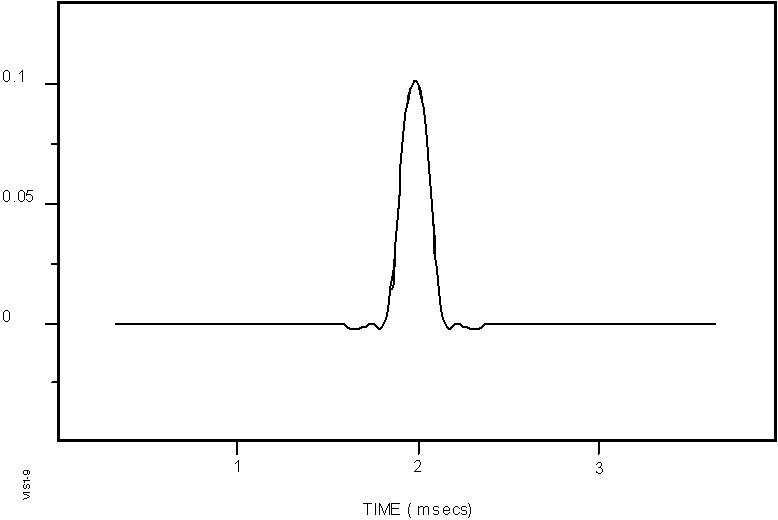Figure 1-9 Autocorrelation Function of the Complementary Series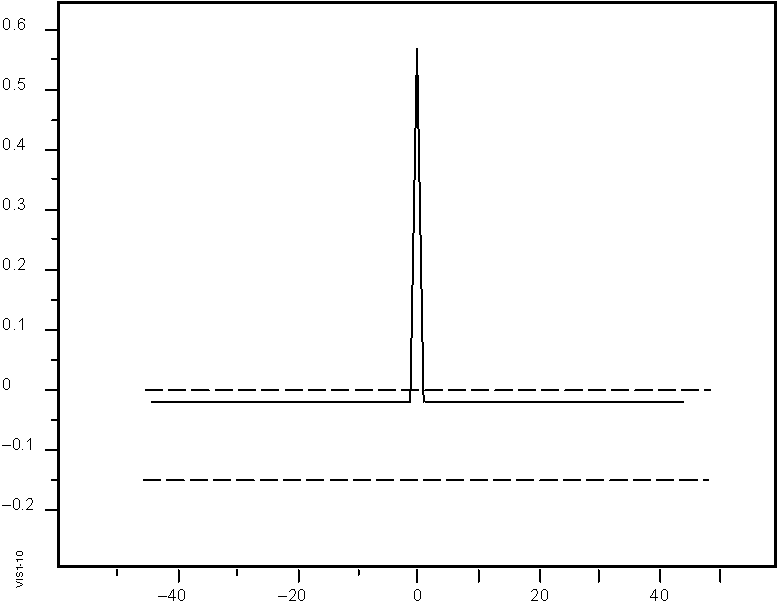Figure 1-10 Autocorrelation Function of a Periodic Maximal Length Sequence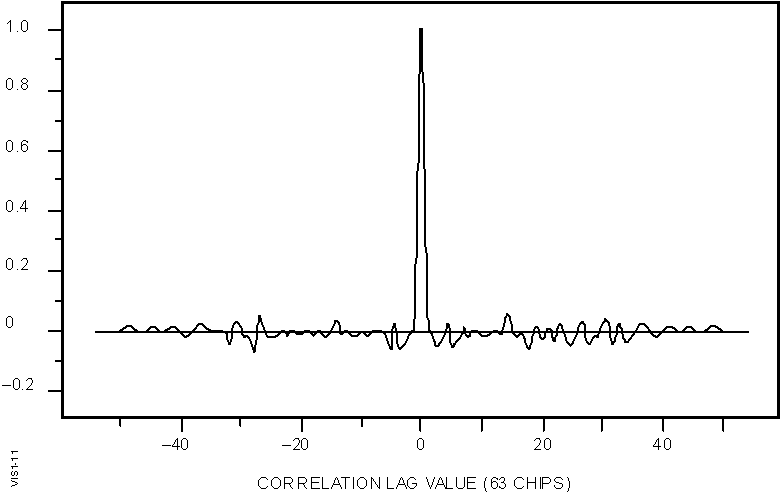Figure 1-11 Autocorrelation Function of a Non-Periodic Maximal Length Sequence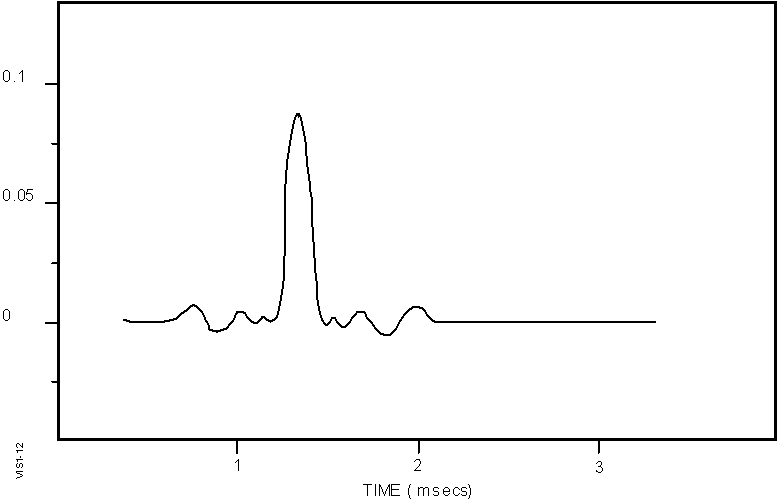Figure 1-12 Autocorrelation Function of the Barker Code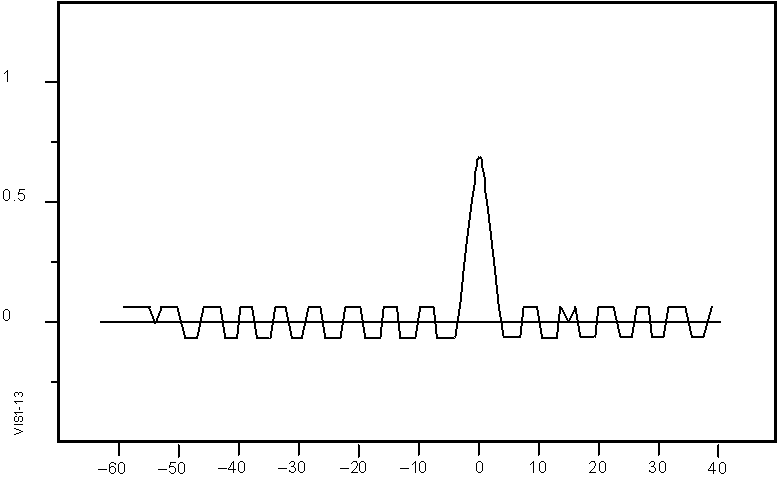Figure 1-13 Autocorrelation Function of the Kasami Sequence

Since the Complementary Series pairs do not leak energy into any other height bin this phase code scheme seemed optimum and was chosen for the DPS’s vertical incidence measurement mode in order to provide the maximum possible dynamic range in the measurement. If there is too much leakage (for instance at a –20 dB level) then stronger echoes would create a "leakage noise floor" in which weaker echoes could not be detectable. The autocorrelation function of the Maximal Length Sequence (M-code) is particularly good since for M = 127, the leakage level is over 40 dB lower than the correlation peak and the correlation peak provides over 20 dB of SNR enhancement. However, since these must be implemented as a continuous transmission (100% duty cycle) they are not suitable for vertical incidence monostatic sounding. Therefore the M-Code is the code of choice for oblique incidence bi-static sounding, where the transmitter need not be shut off to provide a listening interval.

The M-codes which provide the basic structure of the oblique waveform, all have a length of M = (2N–1). The attractive property of the M-codes is their autocorrelation function, shown in Figure 1-10. This type of function is often referred to as a "thumbtack". As long as the code is repeated at least a second time, the value of the cross correlation function at lag values other than zero is –1 while the value at zero is M. However, if the M-Code is not repeated a second time, i.e., if it is a pulsed signal with zero amplitude before and after the pulse, the correlation function looks more like Figure 1-11. The characteristics of Figure 1-11 also apply if the second repetition is modulated in phase, frequency, amplitude, code # or time shift (i.e., starting chip). So to achieve the "clean" correlation function with M-Codes (depicted in Figure 1-10), the identical waveform must be cyclically repeated (i.e., periodic).

The problem that occurs using the M-codes is if any of the multipath signal components starts or ends during the acquisition of one code record, then there are zero amplitude samples (for that multipath component) in the matched filter as the code is being pulse compressed. If this happens then the imperfect cancellation of code amplitude (which is illustrated by Figure 1-11) at correlation lag values other than zero will occur. In order to obtain the thumbtack pulse compression, the matched filter must always be filled with samples from either the last code repetition, the current code repetition or the next code repetition (with no significant change), since these sample values are necessary to make the code compression work. "Priming" the channel with 5 msec of signal before acquiring samples at the receiver ensures that all of the multipath components will have preceding samples to keep the matched filter loaded. Similarly after the end of the last code repetition an extra code repetition makes the synchronization less critical.

This "priming" becomes costly however, for when it is desired to switch frequencies, antennas, polarizations etc., the propagation path(s) have to be primed again. The 75% duty cycle waveform (X = 3) allows these multiplexed operations to occur, but as a result, only 8.5 msec out of each 20 msec of measurement time is spent actually sampling received signals. The 100% duty cycle waveform (X = 4) does not allow multiplexed operation, except that it will perform an O polarization coherent integration time (CIT) immediately after an X polarization CIT has been completed. Since the simultaneity of the O/X multiplexed measurement is not so critical (the amplitude of these two modes fade independently anyway), this is essentially still a simultaneous measurement. Because the 100% mode performs an entire CIT without changing any parameters, it can continuously repeat the code sequence and therefore the channel need only be primed before sampling the very first sample of each CIT. After this subsequent code repetitions are primed by the previous repetition.

Even though the Complementary Code pairs are theoretically perfect, the physical realization of this signal may not be perfect. The Complementary Code pairs achieve zero leakage by producing two compressed pulses (one from each of the two codes) which have the same absolute amplitude spurious correlation peaks (or leakage) at each height, but all except the main correlation peak are inverted in phase between the two codes. Therefore, simply by adding the two pulse compression outputs, the leakage components disappear. Since the technique relies on the phase distance of the propagation path remaining constant between the sequential transmission of the two coded pulses, the phase change vs. time caused by any movement in the channel geometry (i.e., Doppler shift imposed on the signal) can cause imperfect cancellation of the two complex amplitude height profile records. Therefore, the Complementary Code is particularly sensitive to Doppler shifts since channel induced phase changes which occur between pulses will cause the two pulse compressions to cancel imperfectly, while with most other codes we are only concerned with channel induced phase changes within the duration of one pulse. However, if given the parameters of the propagation environment, we can calculate the maximum probable Doppler shift, and determine if this yields acceptable results for vertical incidence sounding.

With 200 pps, the time interval between one pulse and the next is 5 msec. If one pulse is phase modulated with the first of the Complementary Codes, while the next pulse has the second phase code, the interval over which motions on the channel can cause phase changes is only 5 msec. The degradation in leakage cancellation is not significant (i.e., less than –15 dB) until the phase has changed by about 10 degrees between the two pulses. The Doppler induced phase shift is:

where fD is the Doppler shift in Hz and T is the time between pulses.

The Doppler shift can be calculated as:

fD=(f0vr)/c<     (or for a 2-way radar propagation path)
fD=(2f0vr)/c                                     (1-13)

where f0 is the operating frequency and vr is the radial velocity of the reflecting surface toward or away from the sounder transceiver. The radial velocity is defined as the projection of the velocity of motion (v) on the unit amplitude radial vector (r) between the radar location and the moving object or surface, which in the ionosphere is an isodensity surface. This is the scalar product of the two vectors:

vr=v.r=|v|cos(q)                                 (1-14)

A phase change of 10° in 5 msec would require a Doppler shift of about 5.5 Hz, or 160 m/sec radial velocity (roughly half the speed of sound), which seldom occurs in the ionospheric except in the polar cap region. The 8-chip complementary phase code pulse compression and coherent summation of the two echo profiles provides a 16-fold increase in signal amplitude, and a 4-fold increase in noise amplitude for a net signal processing gain of 12 dB. The 127-chip Maximal Length Sequence provides a 127-fold increase in amplitude and a net signal processing gain of 21 dB. The Doppler integration, as described later can provide another 21 dB of SNR enhancement, for a total signal processing gain of 42 dB, as shown by the following discussion.

The pulse compression described above occurs with each pulse transmitted, so the 12 to 21 dB SNR improvement (for 8-bit complementary phase codes or 127-bit M-codes respectively) is achieved without even sending another pulse. However, if the measurement can be repeated phase coherently, the multiple returns can be coherently integrated to achieve an even more detectable or "cleaner" signal. This process is essentially the same as averaging, but since complex signals are used, signals of the same phase are required if the summation is going to increase the signal amplitude. If the phase changes by more than 90° during the coherent integration then continued summation will start to decrease the integrated amplitude rather than increase it. However, if transmitted pulses are being reflected from a stationary object at a fixed distance, and the frequency and phase of the transmitted pulses remain the same, then the phase and amplitude of the received echoes will stay the same indefinitely.

The coherent summation of N echo signals causes the signal amplitude, to increase by N, while the incoherent summation of the noise amplitude in the signal results in an increase in the noise amplitude of only \/N. Therefore with each N pulses integrated, the SNR increases by a factor of \/N in amplitude which is a factor of N in power. This improvement is called signal processing gain and can be defined best in decibels (to avoid the confusion of whether it is an amplitude ratio or a power ratio) as:

Processing Gain = 20 log10 {(Sp/Qp)/ (Si/Qi)}                  (1-15)

where Si is the input signal amplitude, Qi the input noise amplitude, Sp the processed signal amplitude, and Qp the processed noise amplitude. Q is chosen for the random variable to represent the noise amplitude, since N would be confusing in this discussion. This coherent summation is similar to the pulse compression processing described in the preceding section, where N, the number of pulses integrated is replaced by M, the number of code chips integrated.

Another perspective on this process is achieved if the signal is normalized during integration, as is often done in an FFT algorithm to avoid numeric overflow. In this case Sp is nearly equal to Si, but the noise amplitude has been averaged. Thus by invoking the central limit theorem [Freund, 1967 or any basic text on probability], we would expect that as long as the input noise is a zero mean (i.e., no DC offset) Gaussian process, the averaged RMS noise amplitude, snp (p for processed) will approach zero as the integration progresses, such that after N repetitions:

snp2=sni2/N      (the variance represents power)             (1-16)

Since the SNR can be improved by a variable factor of N, one would think, we could use arbitrarily weak transmitters for almost any remote sensing task and just continue integrating until the desired signal to noise ratio (SNR) is achieved. In practical applications the integration time limit occurs when the signal undergoes (or may undergo, in a statistical sense) a phase change of 90°. However, if the signal is changing phase linearly with time (i.e., has a frequency shift, Dw ), the integration time may be extended by Doppler integration (also known as, spectral integration, Fourier integration, or frequency domain integration). Since the Fourier transform applies the whole range of possible phase shifts needed to keep the phase of a frequency shifted signal constant, a coherent summation of successive samples is achieved even though the phase of the signal is changing. The unity amplitude phase shift factor, e–jwt, in the Fourier Integral (shown as Equation 1–17) varies the phase of the signal r(t) as a function of time during integration. At the frequency (w) which stabilizes the phase of the component of r(t) with frequency w over the interval of integration (i.e., makes r(t) e–jwt coherent) the value of the integral increases with time rather than averaging to zero, thus creating an amplitude peak in the Doppler spectrum at the Doppler line which corresponds to w:

F[r(t)]=R(w)=òr(t)e-jwtdt                      (1-17)

Does this imply that an arbitrarily small transmitter can be used for any remote sensing application, since we can just integrate long enough to clearly see the echo signal? To some extent this is true. There is no violation of conservation of energy in this concept since the measurement simply takes longer at a lower power; however, in most real world applications, the medium or environment will change or the reflecting surface will move such that a discontinuous phase change will occur. Therefore a system must be able to detect the received signal before a significant movement (e.g., a quarter to a half of a wavelength) has taken place. This limits the practical length of integration that will be effective.

The discrete time (sampled data) processing looks very similar (as shown in Equation 1–18). For a signal with a constant frequency offset (i.e., phase is changing linearly with time) the integration time can be extended very significantly, by applying unity amplitude complex coefficients before the coherent summation is performed. This stabilizes the phase of a signal which would otherwise drift constantly in phase in one direction or the other (a positive or negative frequency shift), by adding or subtracting increasingly larger phase angles from the signal as time progresses. Then when the phase shifted complex signal vectors are added, they will be in phase as long as that set of "stabilizing" coefficients progress negatively in phase at the same rate as the signal vector is progressing positively. The Fourier transform coefficients serve this purpose since they are unity amplitude complex exponentials (or phasors), whose only function is to shift the phase of the signal, r(n), being analyzed.

Since the DigisondeTM sounders have always done this spectral integration digitally, the following presentation will cover only discrete time (sampled data rather than continuous signal notation) Fourier analysis.

N
F[r(t)]=R[k]=S r[n]exp[-jnk2p/N]             (1-18)
n=0

where r[n] is the sampled data record of the received signal at one certain range bin, n is the pulse number upon which the sample r[n] was taken, T is the time period between pulses, N is the number of pulses integrated (number of samples r[n] taken), and k is the Doppler bin number or frequency index. Since a Doppler spectrum is computed for each range sampled, we can think of the Fourier transforms as F56[w] or F192[w] where the subscripts signify with which range bin the resulting Doppler spectra are associated.

By processing every range bin first by pulse compression (12 to 21 dB of signal processing gain) then by coherent integration, all echoes from each range have gained 21 to 42 dB of processing gain (depending on the waveform used and the length of integration) before any attempt is made to detect them.

NOTE

Further explanation of Equation 1–18 which can be gathered from any good reference on the Discrete Fourier Transformation, such as [Openheim & Schaefer, Prentice Hall, 1975], follows. The total integration time is NT, where T is the sampling period (in the DPS, the time period between transmitted pulses). The frequency spacing between Doppler lines, i.e., the Doppler resolution, is 2p/NT rads/sec (or 1/NT Hz) and the entire Doppler spectrum covers 2p/T rad/sec (with complex input samples this is ± p/T, but with real input samples the positive and negative halves of the spectra are mirror image replicas of each other, so only p/T rad/sec are represented).

What is coherently integrated by the Fourier transformation in the DPS (as in any pulse-Doppler radar) is the time sequence of complex echo amplitudes received at the same range (or height) that is, at the same time delay after each pulse is transmitted. Figure 1-14 shows memory buffers with range or time delay vertically and pulse number (typically 32 to 128 pulses are transmitted) horizontally which hold the received samples as they are acquired by the digitizer. After each pulse is transmitted, one column is filled from the bottom up at regular sampling intervals, as the echoes from progressively higher heights are received (33.3 msec/5 km). These columns of samples are referred to as height profiles, which are not to be confused with electron density profiles, but rather mirror the radar terminology of a "slant range profile" (range becomes height for vertical incidence sounding) which is simply the time record of echoes resulting from a transmitted pulse. A height profile is simply a column of numeric samples which may or may not represent any reflected energy (i.e., they may contain only noise)

.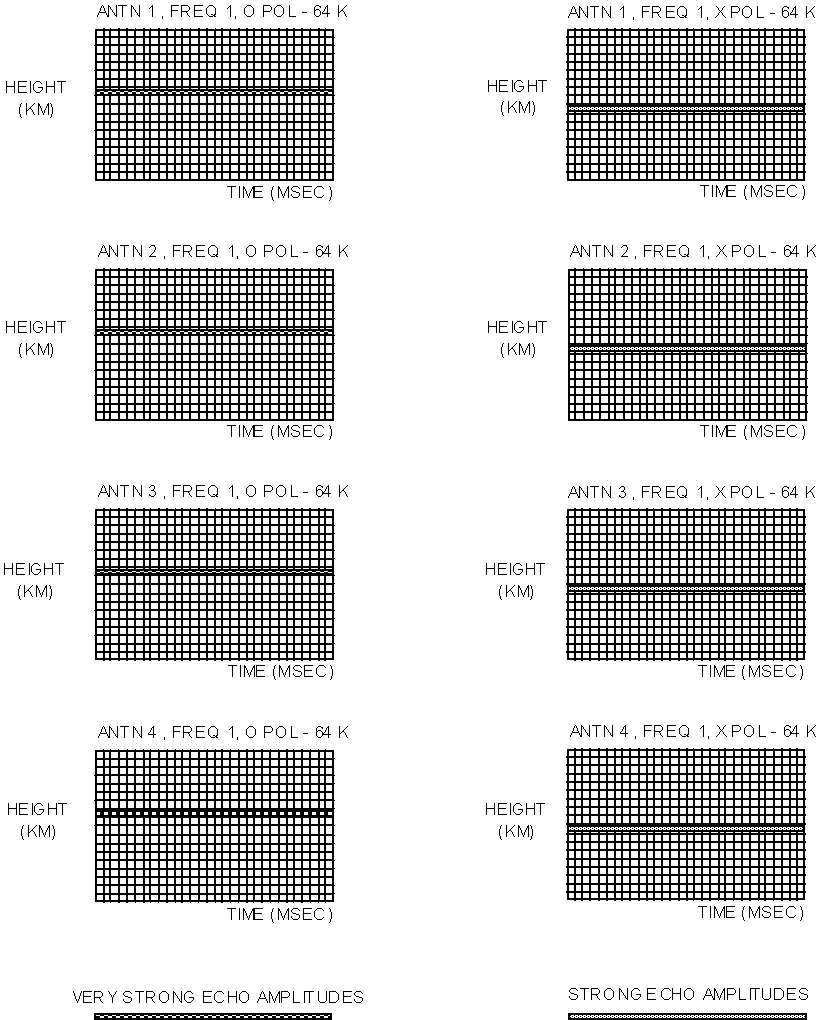Figure 1-14 Eight Coherent Parallel Buffers for Simultaneous Integration of Spectra

With T, the sampling period between subsequent samples of the same coherent process, i.e., the same hardware parameters) defined by the measurement program, the first element of the Discrete Fourier Transform (i.e., the amplitude of the DC component) will have a spectral width of 1/NT. This spectral resolution may be so wide that all Doppler shifts received from the ionosphere fall into this one line. For instance, in the mid-latitudes it is very rare to see Doppler shifts of more that 3 Hz, yet with a ± 50 Hz spectrum of 16 lines, the Doppler resolution is 6.25 Hz, so a 3 Hz Doppler shift would still appear to show "no movement". For sounding, it would be much more interesting if instead of a DC Doppler line, a +3.25 Hz and a –3.25 Hz line were produced, such that even very fine Doppler shifts would indicate whether the motion was up or down. The DC line is a seemingly unalterable characteristic of the FFT method of computing the Discrete Fourier Transform, yet with a true DFT algorithm the Fourier transform coefficients can be chosen such that, the centre of the Doppler lines analyzed can be placed wherever the designer desires them to be. Since the DSP could no longer keep up with the real-time operation if the DFT algorithm were used another solution had to be found. What was needed was a –½ Doppler line shift which would be correct for any value of N or T.

Because the end samples in the sampled time domain function are random, a tapering window had to be used to control the spurious response of the Doppler spectrum to below –40 dB (to keep the SNR high enough to not degrade the phase measurement beyond 1°). Therefore a Hanning function, H(n), which is a real function, was chosen and implemented early in the DPS development. The reader is referred to [Oppenheim and Schafer, 1975] for the definition and applications of the Hanning function. The solution to achieving the ½ Doppler line shift was to make the Hanning function amplitudes complex with a phase rotation of 180° during the entire time domain sampling period NT. The new complex Hanning weighting function is applied simply by performing complex rather than real multiplications. This implements a single-sideband frequency conversion of ½ Doppler line before the FFT is performed. In the following equation, each received multipath signal has only one spectral component (k = Di) such that it can be represented as, ai exp[j2pnDi]:

P
r(n) = {Saiexp[-j2p(nDi)} |H(n)| exp[-j2p(n/2NT)]=
i=1
P
=|H(n)| S ai exp[-j2p(nDi+n/2NT)          (1-19)
i=1

When sending the next pulse, it need not be transmitted at the same frequency, or received on the same antenna with the same polarization. With the DPS it is possible to "go off" and measure something else, then come back later and transmit the same frequency, antenna and polarization combination and fill the second column of the coherent integration buffer, as long as the data from each coherent measurement is not intermingled (all samples integrated together must be from the same coherent statistical process). In this way, several coherent processes can be integrated at the same time. Figure 1-14 shows eight coherent buffers, independently collecting the samples for two different polarizations and four antennas. This can be accomplished by transmitting one pulse for each combination of antenna and polarization while maintaining the same frequency setting (to also integrate a second frequency would require eight more buffers), in which case, each subsequent column in each array will be filled after each eight pulses are transmitted and received. This multiplexing continues until all of the buffers are filled with the desired number of pulse echo records. The DPS can keep track of 64 separate buffers, and each buffer may contain up to 32 768 complex samples. The term "pulse" is used generically here. For Complementary Coded waveforms a pulse actually requires two pulses to be sent, and for 127 chip M-codes the pulse becomes a 100% duty cycle, or CW, waveform. However, in both cases, after each pulse compression, one complex amplitude synthesized pulse, r2(n) in Equation 1–9 which is equivalent to a 67 msec rectangular pulse exists which can be placed into the coherent buffer.

The full buffers now contain a record of the complex amplitude received from each range sampled. Most of these ranges have no echo energy; only externally generated manmade and natural noise or interference from radio transmitters. If a particular ionospheric layer is providing an echo, each height profile will have significant amplitude at the height corresponding to that layer. By Fourier transforming each row of the coherent buffer a Doppler spectrum describing the radial velocity of that layer will be produced. Notice that the sampling frequency at that layer is less than or equal to the pulse repetition frequency (on the order of 100 Hz).

After the sequence of N pulses is processed, the pulse compression and Doppler integration have resulted in a Doppler spectrum stored in memory on the DSP card for each range bin, each antenna, each polarization, and each frequency measured (maximum of 4 MILLION simultaneously integrated samples). The program now scans through each spectrum and selects the largest one amplitude per height. This amplitude is converted to a logarithmic magnitude (dB units) and placed into a new one-dimensional array representing a height profile containing only the maximum amplitude echoes. This technique of selecting the maximum Doppler amplitude at each height is called the modified maximum method, or MMM. If the MMM height profile array is plotted for each frequency step made, this results in an ionogram display, such as the one shown in Figure 1-15.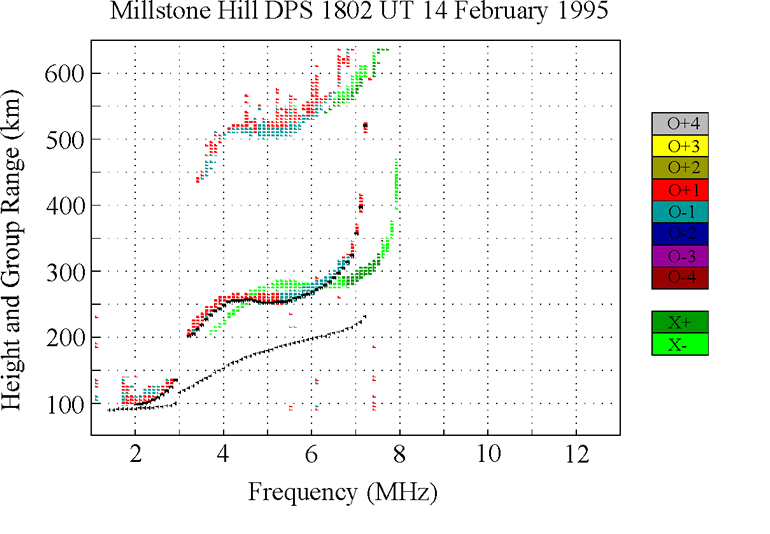Figure 1-15 VI Ionogram Consisting of Amplitudes of Maximum Doppler Lines

Figure 1-16 Angle of Arrival Interferometry

The DPS system uses two distinct techniques for determining the angle of arrival of signals received on the four antenna receiver array, an aperture resolution technique using digital beamforming (implemented as an on-site real-time capability) and a super-resolution technique which is accomplished when the measurement data is being analyzed, in post-processing. Both techniques utilize the basic principle of interferometry, which is illustrated in Figure 1-16. This phenomenon is based on the free space path length difference between a distant source and each of some number of receiving antennas. The phase difference (Df) between antennas is proportional to this free space path difference (Dl) based on the fraction of a wavelength represented by Dl.

Dl=dsinq                                           and
Df=(2pDl)/l=(2p d sinq)/l                      (1-20)

where q is the zenith angle, d is the separation between antennas in the direction of the incident signal (i.e., in the same plane as q is measured), and l is the free space wavelength of the RF signal. This relationship is used to compute the phase shifts required to coherently combine the four antennas for signals arriving in a given beam direction, and this relationship (solved for q) is also the basis of determining angle of arrival directly from the independent phase measurements made on each antenna.

Figure 1-17 shows the physical layout of the four receiving antennas. The various separation distances of 17.3, 34.6, 30 and 60 m are repeated in six different azimuthal planes (i.e., there is six way symmetry in this array) and therefore, the Df ’s computed for one direction also apply to five other directions. This six-way symmetry is exploited by defining the six azimuthal beam directions along the six axes of symmetry of the array, making the beamforming computations very efficient. Section 3 of this manual contains detailed information for the installation of receive antenna arrays.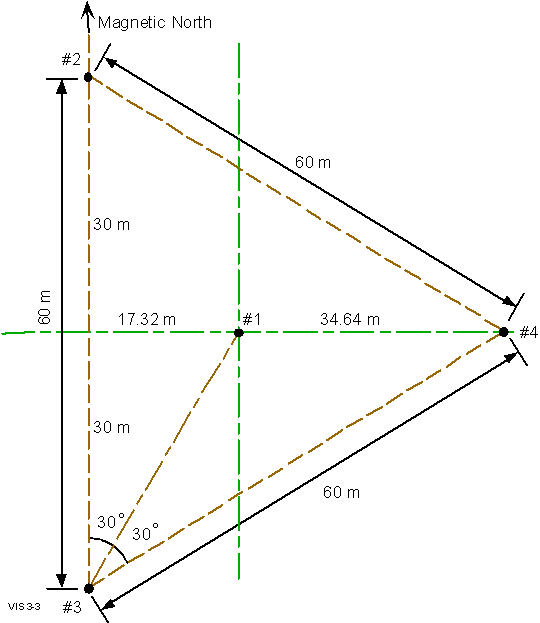Figure 1-17 Antenna Layout for 4-Element Receiver Antenna Array

At the end of the previous section it was shown that after completing a multiplexed coherent integration there is an entire Doppler spectrum stored for each height, each antenna, each frequency and each polarization measured. All of these Doppler lines are available to the beamforming algorithm. In addition, the DSP software stores the complex amplitudes of the maximum Doppler line at each height (i.e., the height profile in an MMM format, is an array of 128 or 256 heights) separately for each antenna. By setting a threshold (typically 6 dB above the noise floor), the heights containing significant echo amplitude can quickly be determined. These are the heights for which beam amplitudes will be computed and a beam direction (the beam which creates the largest amplitude at that height) declared. Due to spatial decorrelation (an interference pattern across the ground) of the signals received at the four antennas, it is possible that the peak amplitude in each of the four Doppler spectra will not appear in the same Doppler line. Therefore, to ensure that the same Doppler line is used for each antenna (using different Doppler lines would negate the significance of any phase difference seen between antennas) only Antenna #1’s spectra are used to determine which Doppler line position will be used for beamforming at each height processed.

At each height where an echo is strong enough to be detected, the four complex amplitudes are passed to a C function (beam_form) where seven beams are formed by phase shifting the four complex samples to compensate for the additional path length in the direction of each selected beam. If a signal has actually arrived from near the centre of one of the beams formed, then after the phase shifting, all four signals can be summed coherently, since they now have nearly the same phase, so that the beam amplitude of the sum is roughly four times each individual amplitude. The farther the true beam direction is away from a given beam centre the farther the phase of the four signals drift apart and the smaller the summed amplitude. However, in the DPS system the beams are so wide that even at the higher frequencies the signal azimuth may deviate more than 30° from the beam centres and the four amplitudes will still sum constructively [Murali, 1993].

The technique for finding the angle of arrival is then simply to compare the amplitude of the signal on each beam and declare the direction as the beam centre of the strongest beam. Therefore the accuracy of this technique is limited to 30° in azimuth and 15° in elevation angle (the six azimuth beams are separated by 60° and the oblique beams are normally set 30° away from the vertical beam); as opposed to the Drift angle of arrival technique described in the next section which obtains accuracies approaching 1°. There may be some question about the amplitude of the sidelobes of these beams, but it is really immaterial (computation of the array pattern for 10 MHz is shown in [Murali, 1993]). The fundamental principle of this technique is that there is no direction which can create a larger amplitude in a given beam than the direction of the centre of that beam. Therefore, detecting the direction by selecting the beam with the largest amplitude can never be an incorrect thing to do. One has to avoid thinking of the beam as excluding echoes from other directions and realize that all that is needed is that a beam favours echoes more as their angle of arrival becomes closer to the centre of that beam. In fact with a four element array the summed amplitude in a wrong direction may be nearly as strong as it is in the correct beam, however, given that the same four complex amplitudes are used as input it cannot be stronger.

The DPS forms seven beams, one overhead (0° zenith angle) and six oblique beams (the nominal 30° zenith angle can be changed by the operator) centred at North and South directions and each 60° in between. Using the same four complex samples (at one reflection height at a time) seven overlapping beams are formed, one overhead (for which the phase shifting required on each antenna is 0°) and six beams each separated by 60° in azimuth and tipped 30° from vertical. If one of the off-vertical beams is found to produce the largest amplitude, the displayed echo on the ionogram is color coded as an oblique reception.

The phase shifts required to sum echoes into each of the seven beams depend on four variables:

a. the signal wavelength,

b. the antenna geometry (separation distance and orientation),

c. the azimuth angle of arrival, and

d. the zenith angle of arrival.

The antenna weighting coefficients are unity amplitude with a phase which is the negative of the extra phase delay caused by the propagation delay, thereby removing the extra phase delay. The phase delays for antenna is resulting from arrival angle spherical coordinates (qj, fj) which corresponds to the direction of beam j, are described (using Equation 1–20) by the following:

DFij=(2p sinqj/l)d'ij                         (1-21)

where DF ij is the phase difference between antenna i’s signal and antenna 1’s signal, qj is the zenith angle (0 for overhead), and d'ij is the projection of the antenna separation distance (from antenna i to antenna 1) upon the wave propagation direction. The parameter d' is dependent on the antenna positions which can be placed on a Cartesian coordinate system with the central antenna, antenna 1, at the origin and the X axis toward the North and the Y axis toward the West. With this definition the azimuth angle f is 0° for signals arriving from the North and:

d'ij=(xi cos fj+yisinfj)                       (1-22)

Since antenna 1 is defined as the origin, x1 and y1 are always zero, so Df i has to be zero. This makes antenna 1 the phase reference point which defines the phase of signals on the other antennas. The correction coefficients bi are unit amplitude phase conjugates of the propagation induced phase delays:

bij=1.0 Ð DFi(f,xi,yi,qj,fj)=1 Ð -DFij        (1-23)

Because they are frequency dependent, these correction factors must be computed at the beginning of each CIT when the beamforming mode of operation has been selected. A full description as well as some modeling and testing results were reported by [Murali, 1993].

 Example A.:Given the antenna geometry shown in Figure 1-17, at an operating frequency of 4.33 MHz (l = 69.28 m), a beam in the eastward direction and 30° off vertical would, according to Equation 1–20, require a phase shift of 90° on antenna 4, –45° on antennas 2 and 3, and 0° on antenna 1. If an echo is received from that direction it would be received on the four antennas as four complex amplitudes at the height corresponding to the height (or more precisely, the range, since there may be a horizontal component to this distance) of the reflecting source feature. Therefore, a single number per antenna can be analyzed by treating one echo height at a time, and by selecting only one (the maximum) complex Doppler line at that height and that antenna. Assume that the following four complex amplitudes have been receive on a DPS system at, for instance, a height of 250 km. This is represented (in polar notation) as:Antenna 1: 830 Ð 135° Antenna 2: 838 Ð 42° Antenna 3: 832 Ð 182° Antenna 4: 827 Ð 179° To these sampled values add the +90° and –45° phase corrections mentioned above producing:Antenna 1: 830 Ð 135° or –586 + j586Antenna 2: 838 Ð 132° or –561 + j623Antenna 3: 832 Ð 137° or –608 + j567Antenna 4: 827 Ð 134° or –574 + j594East Beam (sum of above) = –2329+j2370 (3329Ð 134.5° in polar form)Since the sum is roughly four times the signal amplitude on each antenna there has been a coherent signal enhancement for this received echo because it arrived from the direction of the beam. It is interesting to note here, that these same four amplitudes could have been phase shifted corresponding to another beam direction in which case they would not add up in-phase. The DPS does this seven times at each height, using the same four samples, then detects which beam results in the greatest amplitude at that height. Of course at a different height another source may appear in a different beam, so the beamforming must be computed independently at each height.

Although the received signal is resolved in range/height before beamforming, the beamforming technique is not dependent on isolating a signal source before performing the angle of arrival calculations. If two sources exist in a single Doppler line then these components (the amplitude of the Doppler line can be thought of as a linear superposition of the two signal components) then some of each of them will contribute to an enhanced amplitude in their corresponding beam direction. Conversely, the Drift technique assumes that the incident radio wave is a plane wave (thus requiring isolation of any multiple sources).

By analyzing the spatial variation of phase across the receiver aperture, using Equation 1–20, the two-dimensional angle of arrival (zenith angle and azimuth angle) of a plane wave can be determined precisely using only three antennas. The term super-resolution applies to the ability to resolve distinct closely spaced points when the physical dimensions (in this case, the 60 m length of one side of the triangular array) of the aperture used is insufficient to resolve them (from a geometric optics standpoint). Therefore, the use of interferometry provides super resolution. This is required for the Drift measurements because the beam resolution achievable with a 60 m aperture at 5 MHz is about 60° , while 5° or better is required to measure plasma velocities accurately. Using beamforming to achieve a 5° angular resolution at 5 MHz would require an aperture dimension of 600 m, which would have to be filled with on the order of 100 receiving antenna elements. Therefore the Drift technique described here is a tremendous savings in system complexity. The Drift mode concept appears at first glance to be similar to the beamforming technique, but it is a fundamentally different process.

The Drift mode depends on a single echo source being isolated such that its phase is not contaminated by another echo (from a different direction but possibly arriving with the same time delay). This technique works amazingly well because at a given time, the overhead ionosphere tends to drift uniformly in the same direction with the same velocity. This means that each off-vertical echo will have a Doppler shift proportional to the radial velocity of the reflecting plasma and to cos a where a is the angle between the position vector (radial vector from the observation site to the plasma structure) and velocity vector of the plasma structure, as presented in Equation 1–14. Therefore, for a uniform Drift velocity the sky can be segmented into narrow bands (e.g., 10’s of bands) based on the value of cos a which correspond to particular ranges of Doppler shifts [Reinisch et al, 1992]. These bands are shown in Figure 1-18 as the hyperbolic dashed lines [Scali, 1993] which indicate at what angle of arrival the Doppler line number should change if the whole sky is drifting at the one velocity just calculated by the DDA program. In other words, the agreement of the Doppler transitions with the boundaries specified by the uniform drift assumption is a test of the validity of the assumption for the particular data being analyzed.

Both isolating the sources of different radial velocities and resolving echoes having different ranges (into 10 km height bins), results in very effective isolation of multiple sources into separate range/Doppler bins. If multiple sources exist at the same height they are usually resolved in the Doppler spectrum computed for that height, because of the sorting effect which the uniform motion has on the radial velocities. If the resolution is sufficient that a range/Doppler bin holds signal energy from only one source, the phase information in this Doppler line can be treated as a sample of the phase front of a plane wave. Even though many coherent echoes have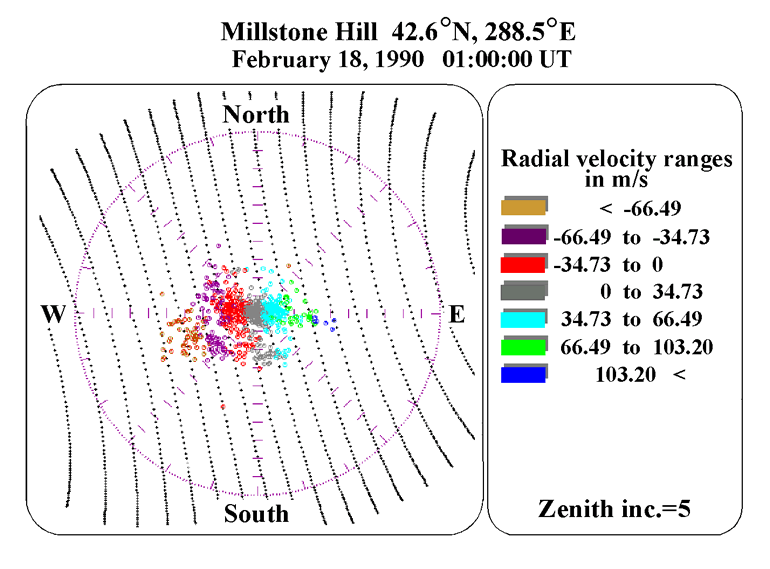Figure 1-18 Radial Velocity Bands as Defined by Doppler Resolution

been received from different points in the sky, the energy from these other points is not represented in the complex amplitude of the Doppler line being processed. This is important because the angle of arrival calculation is accomplished with standard interferometry (i.e., solving Equation 1–20 for q ), which assumes no multiple wave interference (i.e., a perfect plane wave).

A fundamental distinction between the Drift mode and beamforming mode is that in the Drift mode the angle of arrival calculation is applied for each Doppler line in each spectrum at each height sampled, not just at the maximum amplitude Doppler line. A data dependent threshold is applied to try to avoid solving for locations represented by Doppler lines that contain only noise, but even with the threshold applied the resulting angle of arrival map may be filled with echo locations which result from echoes much weaker than the peak Doppler line amplitudes. In beamforming, only the echoes representing the dominant source at each height are stored on tape, therefore no other source echoes are recoverable from the recorded data.

It has been found that vertical velocities are roughly 1/10th the magnitude of horizontal velocities [Reinisch et al, 1991]. Since the horizontal velocities from echoes directly overhead result in zero radial velocity to the station, the Drift technique works best in a very rough, or non-uniform ionosphere, such as that found in the polar cap regions or the equatorial regions, because they provide many off-vertical echoes.

For a smooth spherically concentric (with the surface of the earth) ionosphere all the echoes will arrive from directly overhead and the resulting Drift skymaps will show a single source location at zenith angle = 0°. For horizontal gradients or tilts within that spherically concentric uniform ionosphere however, the single source point would move in the direction of the DN/N (N as in Equation 1–1) gradient (the local electron density gradient), one degree per degree of tilt, so the Drift measurement can provide a straightforward measurement of ionospheric tilt.

Resolution of source components by first isolating multiple echoes in range then in Doppler spread (velocity distribution) combined with interferometer principles is a powerful technique in determining the angle of arrival of superimposed multipath signals.

The phase of an echo from a target, or the phase of a signal after passing through a propagation medium is dependent on three things:

1. the absolute phase of the transmitted signal;

2. the transmitted frequency (or free space wavelength); and

3. the phase distance, d, where:

D
d = ò m(f,x,y,z)dl                             (1-24)
0

is the line integral over the propagation path, scaled by the refractive index if the medium is not free space. If the first two factors, the transmitted phase and frequency, can be controlled very precisely, then measuring the received phase at two different frequencies makes it possible to solve for the propagation distance with an accuracy proportional to the accuracy of the phase measurement, which in turn is proportional to the received SNR. This is often referred to as the df>/df technique. The two measurements form a set of linear equations with two equations and two unknowns, the absolute transmitted phase and the phase distance. If there are several "propagation path distances" as is the case in a multipath environment, then measurement at several wavelengths can provide a measure of each separate distance. However, instead of using a large set of linear equations, the phase of the echoes have chosen to be analyzed as a function of frequency, which can be done very efficiently with a Fast Fourier Transform. The basic relations describing the phase of an echo signal are:

f(f)=-2pftp=-2pd/l=-2p(f/c)d                    (1-25)

where d is the propagation path length in metres (the phase path described in Equation 1–24, f in Hz, f in radians, l in metres and tp is the propagation delay in seconds. Note that the first expression casts the propagation delay in terms of time delay (# of cycles of RF), the second in terms of distance (# of wavelengths of RF), and the third relates frequency and distance using c.

For monostatic radar measurements the distance, d is twice the range, R, so Equation 1–25 becomes:

f(f)=-4pR/l = -4p(f/c)R                       (1-26)

If a series of N RF pulses is transmitted, each changed in frequency by D f, one can measure the phases of the echoes received from a reflecting surface at range R. It is clear from Equation 1–26 that the received phase will change linearly with frequency at a rate directly determined by the magnitude of R. Using Equation 1–26 one can express the received phase from each pulse (indexed by i) in this stepped frequency pulse train:

fi(fi)=-4pfitp=-4pfi(R/c)                      (1-27)

where the transmitted frequency fi can be represented as:

fi=f0 + iDf                                   (1-28)

a start frequency plus some number of incremental steps.

This measurement forms the basis of the DPS’s Precision Group Height mode. By making use of the simultaneous (multiplexed) operation at multiple frequencies (i.e., multiplexing or interlacing the frequency of operation during a coherent integration time ( CIT) it is possible to measure the phases of echoes from a particular height at two different frequencies. If these frequencies are close enough that they are reflected at the same height then the phase difference between the two frequencies determines the height of the echo.

The following development of the two frequency ranging approach leads to a general theory (but not expoused here) covering FM/CW ranging and stepped frequency radar ranging. Using Equation 1–26 a two frequency measurement of f allows the direct computation of R, by:

f2-f1=4pR(f1-f2)/c=4pRDf/c                     (1-29)
R=c(f2-f1)/4pDf                                 (1-30)

It is easy to see from Equation 1–29 that if the range is such that RDf/c is greater than 1/2 then the magnitude of f2-f1 will exceed 2p which is usually not discernible in a phase measurement, and therefore causes an ambiguity. This ambiguity interval (D for distance) is

R=DA=(1/2)c/Df=c/2Df                           (1-31)

 Example B.:The measured phase is (f2 - f1) = p/8 while Df = 1 kHz, then R = 9.375 km.In the example above with Df = 1 kHz, the ambiguous range DA is 150 km. Since a 0 km reflection height must certainly give the same phase for any two frequencies (i.e., 0° ), then given that the ambiguity interval is 150 km, then for this value of Df, the phase difference must again be zero at 150, 300, 450 km etc, since 0 km is one of the equal phase points, and all other ranges giving a phase difference of 0° are spaced from it by 150 km. If the phase measurements f2 and f1 were taken after successive pulses at a time delay corresponding to a range of 160 km (at least one sample of the received echo must be made during each pulse width, i.e., at a rate equal to or greater than the system bandwidth, see Equation 1–4), one would conclude that there is an extra 2p in the phase difference and that the true range is 159.375 km, not 9.375 km. Therefore, the measurement must be designed such that the raw range resolution of the transmitted pulse is sufficient to resolve the ambiguity in the df/df measurement.

The validity of the two-frequency precision ranging technique is lost if there is more than one source of reflection within the resolution of the radar pulse. The phase of the received pulse will be the complex vector sum of the multiple overlapping echoes, and therefore any phase changes (fi) will be partially influenced by each of the multiple sources and will not correctly represent the range to any of them. Therefore, in the general propagation environment where there may be multiple echo sources (objects producing a reflection of RF energy back to the transmitter), or for multipath propagation to and from one or more sources, many frequency steps are needed to resolve the different components influencing fi. This "many step" approach can be performed in discrete frequency steps as in the DPS’s HRR mode, or by a continuous linear sweep, as done in a chirpsounder described in [Haines, 1994].

Signal flow through the DPS Transmitter Exciter

The transmitted code is generated on the transmitter exciter card (XMT) by selecting and clocking out the phase code bits stored in a ROM on the XMT card (Section 5 (Hardware Description) describes the functions of the various system components in detail). These bits are offset and balanced such that their positive and negative swings are equal. Then they are applied to a double balanced mixer along with the 70.08 MHz signal from the oscillator (OSC) card. This multiplication process results in either a 0° or 180° phase inversion since multiplication of a sine wave by –1 is the same as performing a phase inversion, since –sin(t) = sin(t ± p ). This modulated 70.08 MHz signal is then filtered by a linear phase surface acoustic wave (SAW) filter, split into phase quadrature (to enable selection of circular transmitter polarization), and mixed with the variable local oscillator from the Frequency Synthesizer (SYN) card. The mixing process (a passive diode double balanced mixer is used) effectively multiplies the two input signals (along with some non-linear distortion products) which produces a sum and difference frequency at the output:

y(t)=sin(a)sin(b)=0.5[cos(a-b)-cos(a+b)]       (1-32)

The variable local oscillator signal ranges from 71 MHz to 115 MHz, which mixed with 70.08 MHz creates a 1 to 45 MHz difference frequency (a 140 to 185 MHz sum frequency is also produced but is low-pass filtered out of the final signal) which is amplified and sent to the RF power amplifier chassis. The RF amplifier boosts up the signal level to be applied to the antenna(as) for transmission.

The receive loop antennas (Figure 1-1B) are sensitive to the horizontal magnetic field component of the received signal, and can be phased to favour either the right hand circular or left hand circular polarization. The two loop antennas are oriented at a 90° angle to each other and detect the same peak of the incident circularity polarized wave, separated by exactly a quarter of a RF cycle. Therefore, if the phase of the signal on one antenna is shifted by 90° the sum of the two signals has either double the amplitude or zero amplitude depending on the sense of the circular polarization. This is a linear process and therefore treats each of the multipath components independently. For instance if there is one O polarized echo at 250 km and an X polarized echo at 200 km, the fact that the X polarized energy is rejected has no effect on the reception of the O polarized energy. The received signal which is applied to the receivers is the sum of the signals from the two crossed antennas after shifting one by ± 90° with a broadband quadrature phase shifter. The 90° phase shift can be expressed in an equation using the phasor exp[± jp/2], so using the form of Equation 1–6:

P
r(t)=S{aip(t-ti) exp[j2pf0t-jfi]+ai p(t-ti)exp[j2pf0t-
i
-jfi-jp/2] exp[±jp/2]}=
P
=2Saip(t-ti) exp[j2pf0t-jfi] if the last term is exp[+jp/2] OR
i
=0                          if the last term is exp[-jp/2] (1-33)

200 m sec before each waveform is transmitted, the DPS can shift the signal from one of the receive loops either ± 90° under control of the DPS software thus switching sensitivity from left circular polarization to right circular polarization. In the DPS, the signals from the four crossed loop receive antennas are fed into the antenna switch box, which either selects one signal to feed to the single receiver card or combines all four in phase. In the DPS-4 (four-channel receiver variant), one receiver is dedicated to each receive antenna (one receive antenna is the sum of the two crossed elements, but since the two elements are combined in the field and fed to the system on a single coax there is only one signal from each crossed loop assembly). Therefore, in a DPS-4, four signals from the antennas are simply passed through the antenna switch box to the four receivers in which case the only functions of the antenna switch box are to switch in a calibration signal from the transmitter exciter card and to apply the DC power to the receiver antenna preamplifiers via coaxial cables).

The received wideband RF signal from the antenna switch is fed to the receiver (RCV) card where it is first stepped up in voltage 2:1 in a transformer to increase the impedance from 50 to 200 W for a better match to the high input impedance (about 1 kW ) preamplifier. Based on the level of one of the receiver gain control bits, which in turn responds to a manual setting in the DPS hardware setup file (the Hi_Noise parameter) the gain through this amplifier is either 6 dB or 15 dB. Since the maximum achievable output swing from this amplifier is about 8 Vp-p the maximum allowable input voltage is therefore 4 or 1.5 V (at the antenna preamplifier output) respectively for the two different gain settings. Considering the 2:1 step-up, this means that if the wideband input from the receive antennas is over 0.7 Vp-p the lower gain setting must be used. The 8 Vp-p maximum output of the preamplifier is reduced to 5 Vp-p by a 33 W resistor which matches the highest allowed input to the passive diode mixer (the 23 dBm LO level double balanced mixer allows a maximum of 20 dBm input). The remainder of the receiver applies successively more gain and filtering (the bandwidth narrows down to 20 kHz after seven stages of tuning), and outputs the received signal at a fixed 225 kHz intermediate frequency (IF).

The reason for selecting exactly 225 kHz as the last IF frequency is that there are an even number of cycles in the time period that corresponds to a 10 km height interval (66.667 m sec). This means that, if spaced by 66.667 msec, samples of the IF signal (which has a period of 4.444 msec) will represent baseband samples of the received envelope amplitude, since:

15 cycles of 225 kHz=66.6667 msec=10 km radar range.

For instance, if a constant amplitude coherent sine wave carrier were received directly on the current receiver frequency, samples of the IF would have a constant amplitude. The only problem is that without being synchronized to the peaks of this sine wave it is possible that all of the samples of the IF will occur at zero crossings of the received signal. This apparent problem is avoided by the use of quadrature sampling.

The more standard quadrature sampling approach [Peebles, 1979] is to use a 90° phase shifter to produce a quadrature Local Oscillator and down-convert the IF to a complex (two channel) baseband. However, in the DPS since very fast analog to digital (A/D) converters were available inexpensively, the signal was simply sampled as pairs at 90° (1.1111 msec) intervals. This pair of samples is then repeated at the desired sampling interval, 16.6667 msec for 2.5 km delay intervals, 33.3333 msec for 5 km or 66.6667 msec for 10 km intervals [Bibl, K., 1988]. The samples at 2.5 km or 5 km intervals are not equal in phase, since 3.75 and 7.5 cycles respectively have passed between the complex sample pairs. However, at the 10 km interval, exactly 15 cycles have passed. Adjacent 2.5 or 5 km samples within a received pulse should have the same phase since they are sampling the continuation of the coherent transmitted pulse. In order to correct the 90° and 180° phase errors made by the 3.75 or 7.5 cycle sampling interval, an efficient numeric correction brings these samples back into phase. The 90° and 180° phase correction is simply a matter of inverting the sign for 180° or swapping the real and imaginary samples and inverting the real sample for the 90° shift. No complex multiplications are required but this does add another level of "bookkeeping" to the signal processing algorithms.

From here, the next step is to cross-correlate the received samples with the known phase code, as was described in the above section on Coherent Phase Modulation and Pulse Compression. The known phase code is either ± 1 for each code chip, therefore the cross multiplication required in the correlation process is in reality only addition or subtraction. However, with a modern signal processor, the pipelined multiplication process is faster than addition due to the on-chip hardware multiplier and automatic sequencing of address pointers, so as implemented, the multiplications by ± 1. Another interesting detail in this algorithm is that the real samples and the imaginary samples are pulse compressed independently of each other. The two resulting range profiles are then combined into complex samples which represent the phase and amplitude of the original RF signal at the height/range corresponding to the correlation time lag of the cross-correlation function. As is evident from Equation 1–9, this is a linear process and therefore superimposed signals at different time delays can be detected without distorting each other as was shown by Figure 1-8.

Another interesting feature of the DPS’s pulse compression algorithm is a technique to avoid the M2 processing load penalty inherent in the pulse compression operation when the phase code chips are double sampled (5 km sample period, making the pulse duration 16 samples) or quadruple sampled (2.5 km intervals, making the pulse duration 32 samples). Since the phase transitions are always 66.667 msec apart, we can "decimate" the input record by taking every 2nd or 4th sample and then cross-correlating it with an 8-sample matched filter rather than a 16 or 32 sample matched filter. The full 4 times over-sampled resolution can be restored by successively taking each fourth sample but starting one sample higher each time. Then after performing the four cross-correlation functions, interleave the four pulse compressed records back into a new 4 times over-sampled output record. A quantitative analysis of the savings in processing steps is presented next.

When the phase code chips are double sampled (5 km sample period) or quadruple sampled (2.5 km intervals) the M2 increased processing load required for a cross correlation is avoided by independently performing the pulse compression of the odd #’d and even #’d samples (for 5 km spacing, or each fourth sample for 2.5 km sample spacing, since the signal’s range resolution is only 10 km) and reconstructing the finer resolution profile after compression. In addition the savings obtained by processing the real record and imaginary record simultaneously is analyzed. The number of operations required to cross-correlate a 256 sample complex data record (e.g., a 256 sample height profile), using 5 km sampling intervals, and the 127 length maximal-length sequence code are as follows:

1) Cross correlating the 2 times over-sampled record:
256-pt complex record convolved with 254-pt MF       260,096 multiplications

Knowing that the real and imaginary samples are independent and that the phase code itself is all real, the complex multiplications (i.e., the cross-terms) can be done away with, resulting in:

2) Two 256-pt real records convolved with 254-pt MF  130,048 multiplications

By pulse compressing only every other sample in a double over-sampled record then going back and compressing the every other sample skipped the first time:

3) Four 128-pt real records convolved with 127-pt MF  65,024 multiplications

With the much shorter Complementary Codes, the pulse compression computational load is greatly reduced, since only an 8-pt MF is used. Using the same real pulse compression algorithm and skipping every other sample, the Complementary Code processing load is:

4) Eight 128-pt real records (the 8 sub-records are: real and
imaginary samples, odd and even height numbers, then code 1
and code 2) convolved with an 16-pt filter
16,384 multiplications

Implemented in the TMS320C25 16-bit fixed point processor, these pulse compression algorithms run at about 10 000 multiplications and additions (they are done in parallel) per millisecond, so these pulse compressions with 20 msec between repetitions of the 127-length codes and 10 msec between Complementary Code pairs are easily performed in real time (e.g., one waveform is entirely processed before the next waveform repetition is finished).

A faster way to perform the matched filter convolution is described by Oppenheim and Schaefer [Oppenheim & Schaefer, 1976] which uses Fourier transforms. This is based on the Fourier transform identity:

S(w)=F(w)H(w)                      is an identical expression to:

F[s(t)]=F[f(t)*h(t)]                            (1-34)

This identity says that multiplication in the frequency domain accomplishes a convolution in the time domain, if the transformed function (the product of the two functions, S(w) in the Equation 1–34) is transformed back to the time domain. This would reduce the compression of the 127 chip waveform (sampled twice per code chip) from 65 000 operations to about 4500 operations (Nlog2(N) for N=512 points). This algorithm change has not been implemented. To incorporate this algorithm the samples must be doubled again, since the code repeats at an interval other than a power of two, to accommodate the cyclic nature of the convolutional code compression algorithm. Furthermore, the sampling rate must always be 60 000 samples/sec (the 2.5 km resolution mode) to preclude aliasing from under-sampling.

Regardless of how it is performed the Complementary Code pulse compression provides 12 dB of SNR improvement and the M-codes (only useful in a bi-static measurement) provide 21 dB of SNR improvement. In addition to that, the coherent Doppler integration described above provides another 9 to 21 dB of SNR improvement.

The pulse compression and Doppler integration have resulted in a Doppler spectrum stored in memory on the DSP card for each range bin. The program now scans through each spectrum and selects the largest amplitude. This amplitude is converted to a logarithmic magnitude (dB units) and placed into a one-dimensional array representing a time-delay profile of any echoes. This one dimensional array is called a height profile, or range profile, and if plotted for each frequency step made, results in an ionogram display, such as the one shown in Figure 1-17. The 11 520 amplitudes shown as individual pixels on the height vs. frequency display are the amplitude of the maximum Doppler line from the spectrum at each height and frequency. Therefore, the ionogram shown, covering 9 MHz in 100 kHz steps is the result of 737 280 separate samples, and 23 040 separate Doppler spectra (11 520 O polarization and 11 520 X polarization).

BIBLIOGRAPHY

Barker R.H., "Group Synchronizing of Binary Digital Systems", Communication Theory, London, pp. 273-287, 1953

Bibl, K. and Reinisch B.W., "Digisonde 128P, An Advanced Ionospheric Digital Sounder", University of Lowell Research Foundation, 1975.

Bibl, K and Reinisch B.W., "The Universal Digital Ionosonde", Radio Science, Vol. 13, No. 3, pp 519-530, 1978.

Bibl K., Reinisch B.W., Kitrosser D.F., "General Description of the Compact Digital Ionospheric Sounder, Digisonde 256", University of Lowell Center for Atmos Rsch, 1981.

Bibl K., Personal Communication, 1988.

Buchau, J. and Reinisch B.W., "Electron Density Structures in the Polar F Region", Advanced Space Research, 11, No. 10, pp 29-37, 1991.

Buchau, J., Weber E.J. , Anderson D.N., Carlson H.C. Jr, Moore J.G., Reinisch B.W. and Livingston R.C., "Ionospheric Structures in the Polar Cap: Their Origin and Relation to 250 MHz Scintillation", Radio Science, 20, No. 3, pp 325-338, May-June 1985.

Bullett T., Doctoral Thesis, University of Massachusetts, Lowell, 1993.

Chen, F., "Plasma Physics and Nuclear Engineering", Prentice-Hall, 1987.

Coll D.C., "Convoluted Codes", Proc of IRE, Vol. 49, No 7, 1961.

Davies, K., "Ionospheric Radio", IEE Electromagnetic Wave Series 31, 1989.

Golay M.S., "Complementary Codes", IRE Trans. on Information Theory, April 1961.

Huffman D. A., "The Generation of Impulse-Equivalent Pulse Trains", IRE Trans. on Information Theory, IT-8, Sep 1962.

Haines, D.M., "A Portable Ionosonde Using Coherent Spread Spectrum Waveforms for Remote Sensing of the Ionosphere", UMLCAR, 1994.

Hayt, W. H., "Engineering Electromagnetics", McGraw-Hill, 1974.

Murali, M.R., "Digital Beamforming for an Ionospheric HF Sounder", University of Massachusetts, Lowell, Masters Thesis, August 1993.

Oppenheim, A. V., and R. W. Schafer, "Digital Signal Processing", Prentice Hall, 1976.

Peebles, P. Z., "Communication System Principles", Addison-Wesley, 1979.

Reinisch, B.W., "New Techniques in Ground-Based Ionospheric Sounding and Studies", Radio Science, 21, No. 3, May-June 1987.

Reinisch, B.W., Buchau, J. and Weber, E.J., "Digital Ionosonde Observations of the Polar Cap F Region Convection", Physica Scripta, 36, pp. 372-377, 1987.

Reinisch, B. W., et al., "The Digisonde 256 Ionospheric Sounder World Ionosphere/ Thermosphere Study, WITS Handbook, Vol. 2, Ed. by C. H. Liu, December 1989.

Reinisch, B.W., Haines, D.M. and Kuklinski, W.S., "The New Portable Digisonde for Vertical and Oblique Sounding," AGARD-CP-502, February 1992.

Rush, C.M., "An Ionospheric Observation Network for use in Short-term Propagation Predictions", Telecomm, J., 43, p 544, 1978.

Sarwate D.V. and Pursley M.B., "Crosscorrelation Properties of Pseudorandom and Related Sequences", Proc. of the IEEE, Vol 68, No 5, May 1980.

Scali, J.L., "Online Digisonde Drift Analysis", User’s Manual, University of Massachusetts Lowell Center for Atmospheric Research, 1993.

Schmidt G., Ruster R. and Czechowsky, P., "Complementary Code and Digital Filtering for Detection of Weak VHF Radar Signals from the Mesosphere", IEEE Trans on Geoscience Electronics, May 1979.

Wright, J.W. and Pitteway M.L.V., "Data Processing for the Dynasonde", J. Geophys. Rsch, 87, p 1589, 1986.